• 本文原始地址：最大似然估计（Maximum likelihood estimation, 简称MLE）和最大后验概率估计（Maximum a posteriori estimation, 简称MAP）是很常用的两种参数估计方法，如果不理解这两种方法的思路，很
声明：本文为原创文章，发表于nebulaf91的csdn博客。欢迎转载，但请务必保留本信息，注明文章出处。 本文作者: nebulaf91 本文原始地址：http://blog.csdn.net/u011508640/article/details/72815981

最大似然估计（Maximum likelihood estimation, 简称MLE）和最大后验概率估计（Maximum a posteriori estimation, 简称MAP）是很常用的两种参数估计方法，如果不理解这两种方法的思路，很容易弄混它们。下文将详细说明MLE和MAP的思路与区别。
但别急，我们先从概率和统计的区别讲起。
概率和统计是一个东西吗？
概率（probabilty）和统计（statistics）看似两个相近的概念，其实研究的问题刚好相反。
概率研究的问题是，已知一个模型和参数，怎么去预测这个模型产生的结果的特性（例如均值，方差，协方差等等）。 举个例子，我想研究怎么养猪（模型是猪），我选好了想养的品种、喂养方式、猪棚的设计等等（选择参数），我想知道我养出来的猪大概能有多肥，肉质怎么样（预测结果）。
统计研究的问题则相反。统计是，有一堆数据，要利用这堆数据去预测模型和参数。仍以猪为例。现在我买到了一堆肉，通过观察和判断，我确定这是猪肉（这就确定了模型。在实际研究中，也是通过观察数据推测模型是／像高斯分布的、指数分布的、拉普拉斯分布的等等），然后，可以进一步研究，判定这猪的品种、这是圈养猪还是跑山猪还是网易猪，等等（推测模型参数）。
一句话总结：概率是已知模型和参数，推数据。统计是已知数据，推模型和参数。
显然，本文解释的MLE和MAP都是统计领域的问题。它们都是用来推测参数的方法。为什么会存在着两种不同方法呢？ 这需要理解贝叶斯思想。我们来看看贝叶斯公式。
贝叶斯公式到底在说什么？
学习机器学习和模式识别的人一定都听过贝叶斯公式(Bayes’ Theorem)：

P

(

A

∣

B

)

=

P

(

B

∣

A

)

P

(

A

)

P

(

B

)

P(A|B) = \frac{P(B|A)P(A)}{P(B)}

【式1】
贝叶斯公式看起来很简单，无非是倒了倒条件概率和联合概率的公式。
把B展开，可以写成：

P

(

A

∣

B

)

=

P

(

B

∣

A

)

P

(

A

)

P

(

B

∣

A

)

P

(

A

)

+

P

(

B

∣

∼

A

)

P

(

∼

A

)

P(A|B) = \frac{P(B|A)P(A)}{P(B|A)P(A) + P(B|\sim A)P(\sim A)}

【式2】（

∼

A

\sim A

表示"非A"）
这个式子就很有意思了。
想想这个情况。一辆汽车（或者电瓶车）的警报响了，你通常是什么反应？有小偷？撞车了？ 不。。 你通常什么反应都没有。因为汽车警报响一响实在是太正常了！每天都要发生好多次。本来，汽车警报设置的功能是，出现了异常情况，需要人关注。然而，由于虚警实在是太多，人们渐渐不相信警报的功能了。
贝叶斯公式就是在描述，你有多大把握能相信一件证据？（how much you can trust the evidence）
我们假设响警报的目的就是想说汽车被砸了。把A计作“汽车被砸了”，B计作“警报响了”，带进贝叶斯公式里看。我们想求等式左边发生

A

∣

B

A|B

的概率，这是在说警报响了，汽车也确实被砸了。汽车被砸**引起（trigger）**警报响，即

B

∣

A

B|A

。但是，也有可能是汽车被小孩子皮球踢了一下、被行人碰了一下等其他原因（统统计作

∼

A

\sim A

），其他原因引起汽车警报响了，即

B

∣

∼

A

B|\sim A

。那么，现在突然听见警报响了，这时汽车已经被砸了的概率是多少呢（这即是说，警报响这个证据有了，多大把握能相信它确实是在报警说汽车被砸了）？想一想，应当这样来计算。用警报响起、汽车也被砸了这事件的数量，除以响警报事件的数量（这即【式1】）。进一步展开，即警报响起、汽车也被砸了的事件的数量，除以警报响起、汽车被砸了的事件数量加上警报响起、汽车没被砸的事件数量（这即【式2】）。
可能有点绕，请稍稍想一想。
再思考【式2】。想让

P

(

A

∣

B

)

=

1

P(A|B) = 1

，即警报响了，汽车一定被砸了，该怎么做呢？让$P(B|\sim A)P(\sim A) = 0 即 可 。 很 容 易 想 清 楚 ， 假 若 让 即可。很容易想清楚，假若让 P(\sim A) = 0$，即杜绝了汽车被球踢、被行人碰到等等其他所有情况，那自然，警报响了，只剩下一种可能——汽车被砸了。这即是提高了响警报这个证据的说服力。
从这个角度总结贝叶斯公式：做判断的时候，要考虑所有的因素。 老板骂你，不一定是你把什么工作搞砸了，可能只是他今天出门前和太太吵了一架。
再思考【式2】。观察【式2】右边的分子，

P

(

B

∣

A

)

P(B|A)

为汽车被砸后响警报的概率。姑且仍为这是1吧。但是，若

P

(

A

)

P(A)

很小，即汽车被砸的概率本身就很小，则

P

(

B

∣

A

)

P

(

A

)

P(B|A)P(A)

仍然很小，即【式2】右边分子仍然很小，$P(A|B)$ 还是大不起来。 这里，​

P

(

A

)

P(A)

即是常说的先验概率，如果A的先验概率很小，就算

P

(

B

∣

A

)

P(B|A)

较大，可能A的后验概率

P

(

A

∣

B

)

P(A|B)

还是不会大（假设

P

(

B

∣

∼

A

)

P

(

∼

A

)

P(B|\sim A)P(\sim A)

不变的情况下）。
从这个角度思考贝叶斯公式：一个本来就难以发生的事情，就算出现某个证据和他强烈相关，也要谨慎。证据很可能来自别的虽然不是很相关，但发生概率较高的事情。 发现刚才写的代码编译报错，可是我今天状态特别好，这语言我也很熟悉，犯错的概率很低。因此觉得是编译器出错了。 ————别，还是先再检查下自己的代码吧。
好了好了，说了这么多，下面言归正传，说一说MLE。
——————不行，还得先说似然函数（likelihood function）
似然函数
似然（likelihood）这个词其实和概率（probability）是差不多的意思，Colins字典这么解释：The likelihood of something happening is how likely it is to happen. 你把likelihood换成probability，这解释也读得通。但是在统计里面，似然函数和概率函数却是两个不同的概念（其实也很相近就是了）。
对于这个函数：

P

(

x

∣

θ

)

P(x|\theta)

输入有两个：x表示某一个具体的数据；

θ

\theta

表示模型的参数。
如果

θ

\theta

是已知确定的，

x

x

是变量，这个函数叫做概率函数(probability function)，它描述对于不同的样本点x，其出现概率是多少。
如果

x

x

是已知确定的，

θ

\theta

是变量，这个函数叫做似然函数(likelihood function), 它描述对于不同的模型参数，出现x这个样本点的概率是多少。
这有点像“一菜两吃”的意思。其实这样的形式我们以前也不是没遇到过。例如，$f(x, y) = x^y , 即 , 即 x 的 的 y 次 方 。 如 果 次方。如果 x 是 已 知 确 定 的 ( 例 如 是已知确定的(例如 x = 2 ) ， 这 就 是 )，这就是 f(y) = 2^y , 这 是 指 数 函 数 。 如 果 , 这是指数函数。 如果 y 是 已 知 确 定 的 ( 例 如 是已知确定的(例如 y = 2 ) ， 这 就 是 )，这就是 f(x) = x^2$，这是二次函数。同一个数学形式，从不同的变量角度观察，可以有不同的名字。
这么说应该清楚了吧？ 如果还没讲清楚，别急，下文会有具体例子。
现在真要先讲讲MLE了。。
最大似然估计（MLE）
假设有一个造币厂生产某种硬币，现在我们拿到了一枚这种硬币，想试试这硬币是不是均匀的。即想知道抛这枚硬币，正反面出现的概率（记为

θ

\theta

）各是多少？
这是一个统计问题，回想一下，解决统计问题需要什么？ 数据！
于是我们拿这枚硬币抛了10次，得到的数据（

x

0

x_0

）是：反正正正正反正正正反。我们想求的正面概率

θ

\theta

是模型参数，而抛硬币模型我们可以假设是 二项分布。
那么，出现实验结果$x_0$（即反正正正正反正正正反）的似然函数是多少呢？

f

(

x

0

,

θ

)

=

(

1

−

θ

)

×

θ

×

θ

×

θ

×

θ

×

(

1

−

θ

)

×

θ

×

θ

×

θ

×

(

1

−

θ

)

=

θ

7

(

1

−

θ

)

3

=

f

(

θ

)

f(x_0 ,\theta) = (1-\theta)\times\theta\times\theta\times\theta\times\theta\times(1-\theta)\times\theta\times\theta\times\theta\times(1-\theta) = \theta ^ 7(1 - \theta)^3 = f(\theta)

注意，这是个只关于

θ

\theta

的函数。而最大似然估计，顾名思义，就是要最大化这个函数。我们可以画出

f

(

θ

)

f(\theta)

的图像：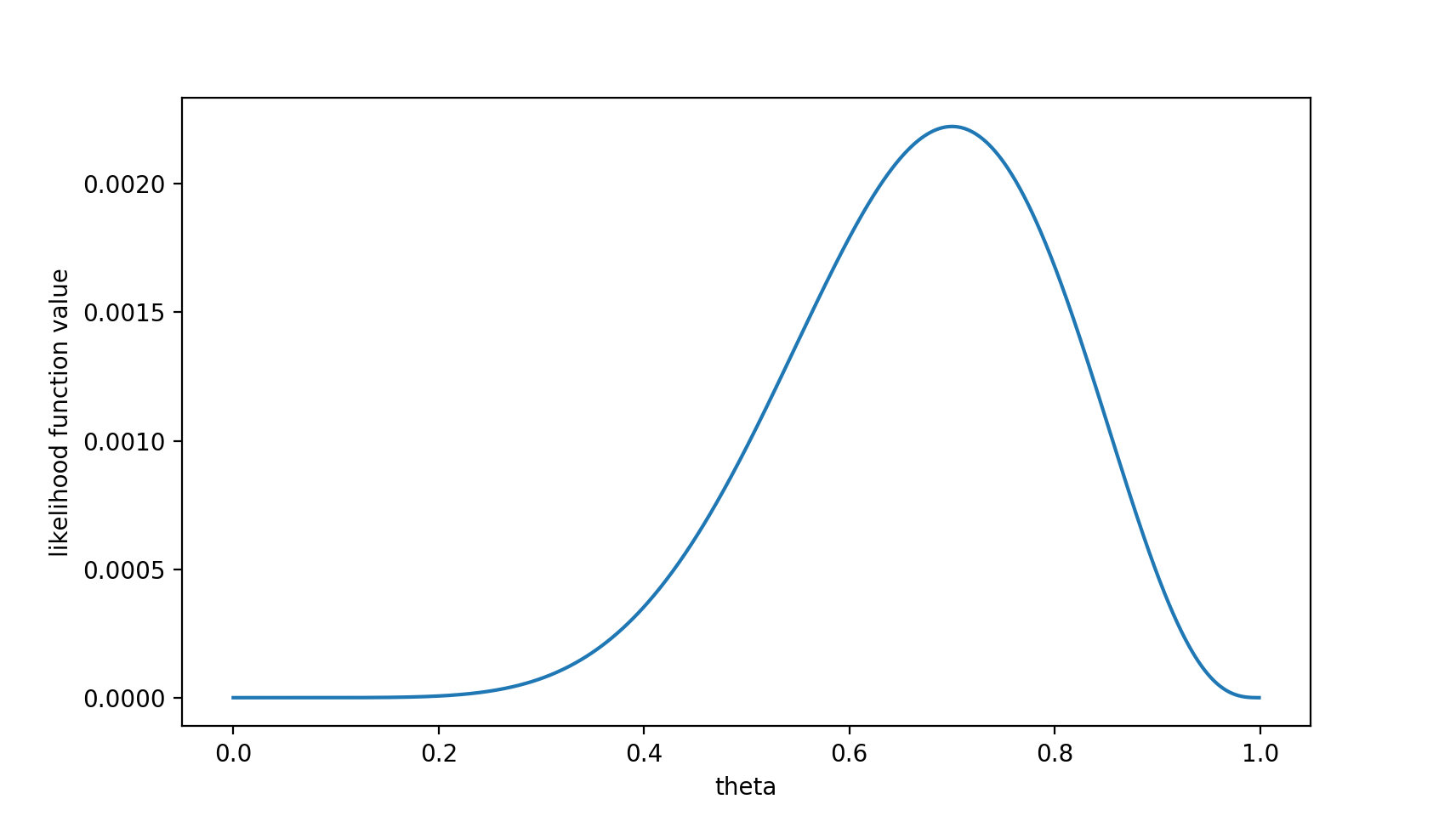可以看出，在

θ

=

0.7

\theta = 0.7

时，似然函数取得最大值。
这样，我们已经完成了对

θ

\theta

的最大似然估计。即，抛10次硬币，发现7次硬币正面向上，最大似然估计认为正面向上的概率是0.7。（ummm…这非常直观合理，对吧？）
且慢，一些人可能会说，硬币一般都是均匀的啊！ 就算你做实验发现结果是“反正正正正反正正正反”，我也不信

θ

=

0.7

\theta = 0.7

。
这里就包含了贝叶斯学派的思想了——要考虑先验概率。 为此，引入了最大后验概率估计。
最大后验概率估计
最大似然估计是求参数

θ

\theta

, 使似然函数$P(x_0 | \theta) 最 大 。 最 大 后 验 概 率 估 计 则 是 想 求 最大。最大后验概率估计则是想求 \theta 使 使 P(x_0 | \theta) P(\theta) 最 大 。 求 得 的 最大。求得的 \theta 不 单 单 让 似 然 函 数 大 ， 不单单让似然函数大， \theta$自己出现的先验概率也得大。 （这有点像正则化里加惩罚项的思想，不过正则化里是利用加法，而MAP里是利用乘法）
MAP其实是在最大化

P

(

θ

∣

x

0

)

=

P

(

x

0

∣

θ

)

P

(

θ

)

P

(

x

0

)

P(\theta|x_0) = \frac{P(x_0|\theta)P(\theta)}{P(x_0)}

，不过因为

x

0

x_0

是确定的（即投出的“反正正正正反正正正反”），

P

(

x

0

)

P(x_0)

是一个已知值，所以去掉了分母

P

(

x

0

)

P(x_0)

（假设“投10次硬币”是一次实验，实验做了1000次，“反正正正正反正正正反”出现了n次，则

P

(

x

0

)

=

n

/

1000

P(x_0) = n/1000

。总之，这是一个可以由数据集得到的值）。最大化

P

(

θ

∣

x

0

)

P(\theta | x_0)

的意义也很明确，

x

0

x_0

已经出现了，要求

θ

\theta

取什么值使

P

(

θ

∣

x

0

)

P(\theta | x_0)

最大。顺带一提，

P

(

θ

∣

x

0

)

P(\theta | x_0)

即后验概率，这就是“最大后验概率估计”名字的由来。
对于投硬币的例子来看，我们认为（”先验地知道“）

θ

\theta

取0.5的概率很大，取其他值的概率小一些。我们用一个高斯分布来具体描述我们掌握的这个先验知识，例如假设

P

(

θ

)

P(\theta)

为均值0.5，方差0.1的高斯函数，如下图：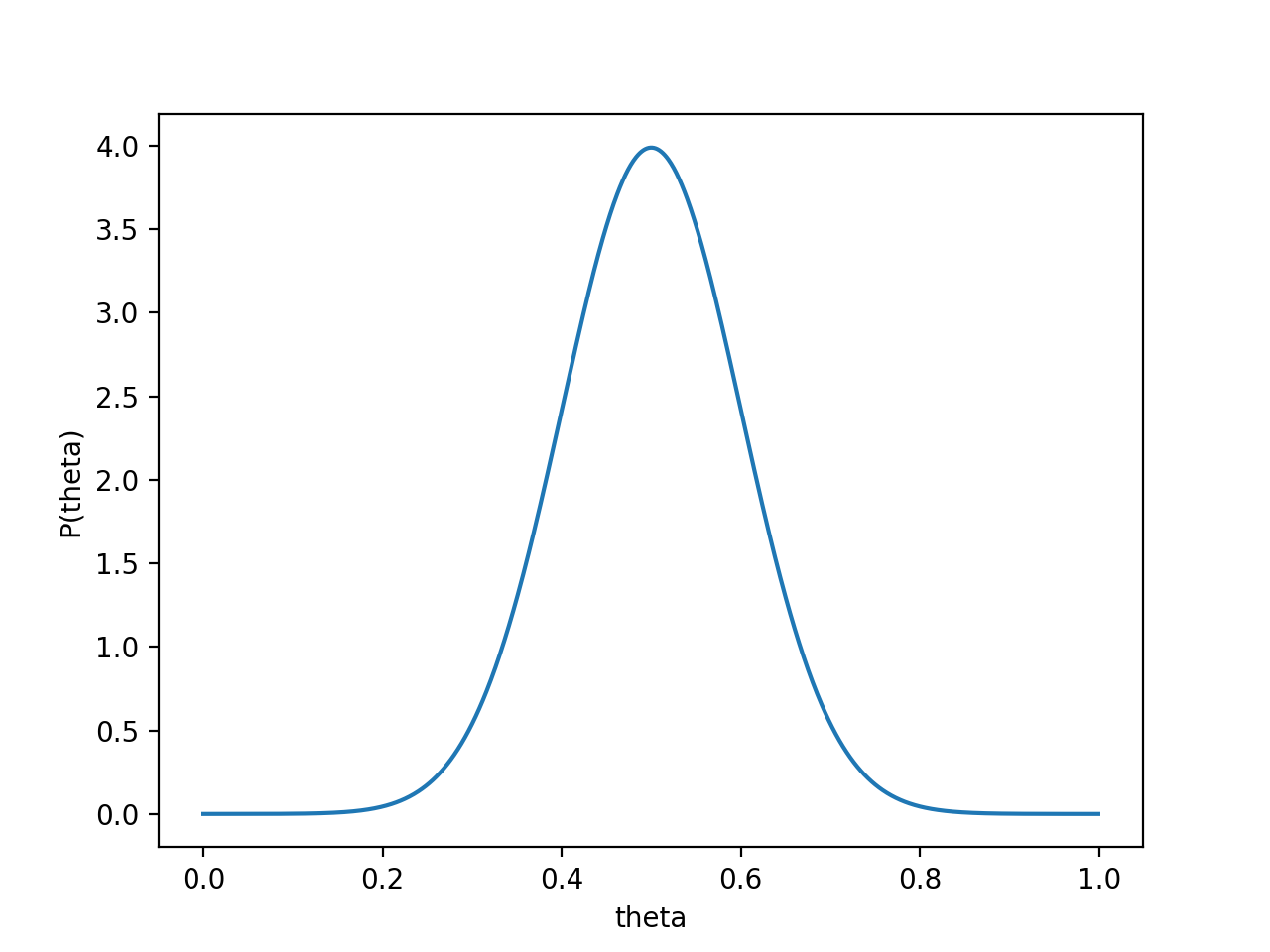则

P

(

x

0

∣

θ

)

P

(

θ

)

P(x_0 | \theta) P(\theta)

的函数图像为：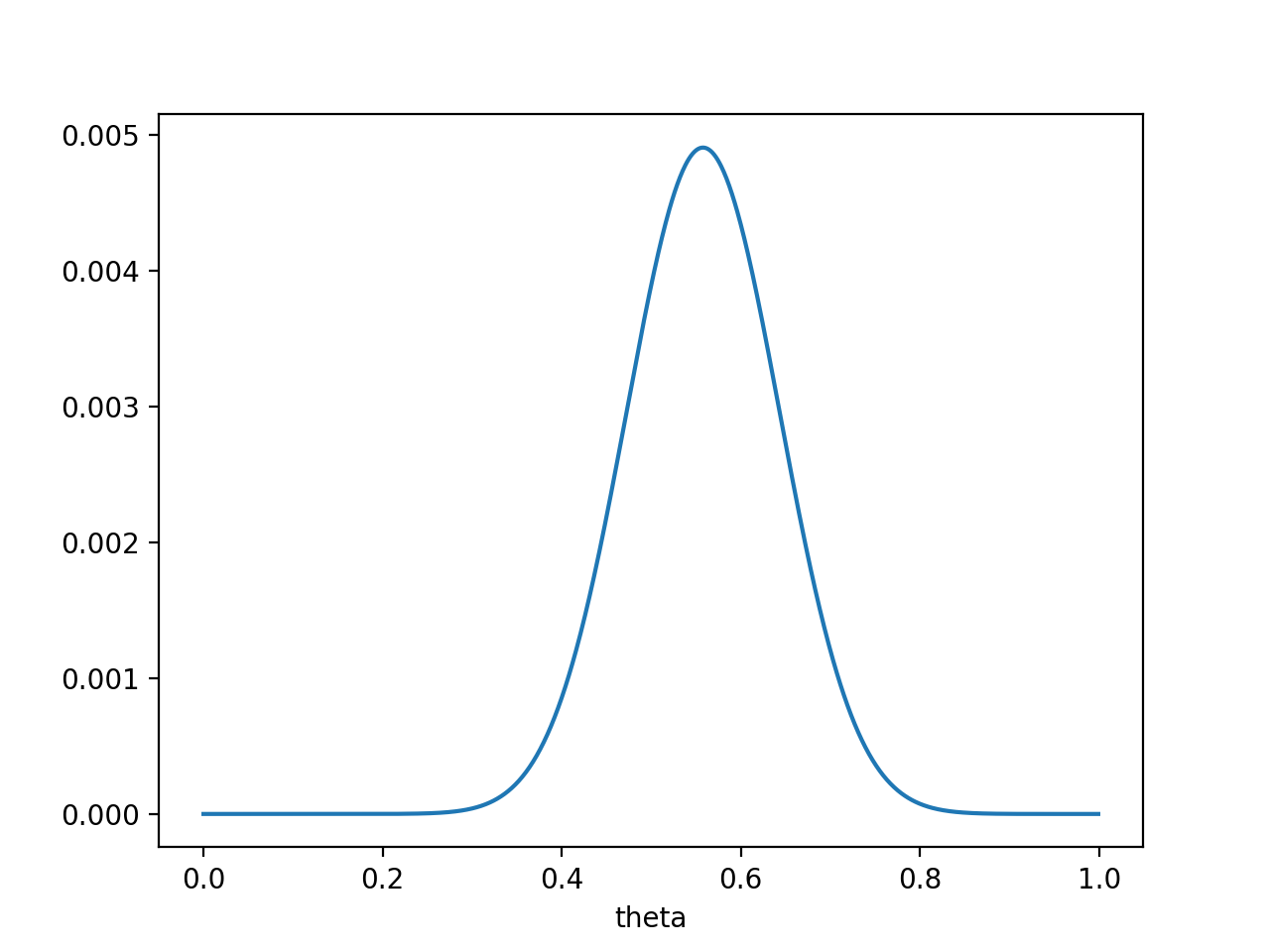注意，此时函数取最大值时，

θ

\theta

取值已向左偏移，不再是0.7。实际上，在

θ

=

0.558

\theta = 0.558

时函数取得了最大值。即，用最大后验概率估计，得到

θ

=

0.558

\theta = 0.558

最后，那要怎样才能说服一个贝叶斯派相信

θ

=

0.7

\theta = 0.7

呢？你得多做点实验。。
如果做了1000次实验，其中700次都是正面向上，这时似然函数为: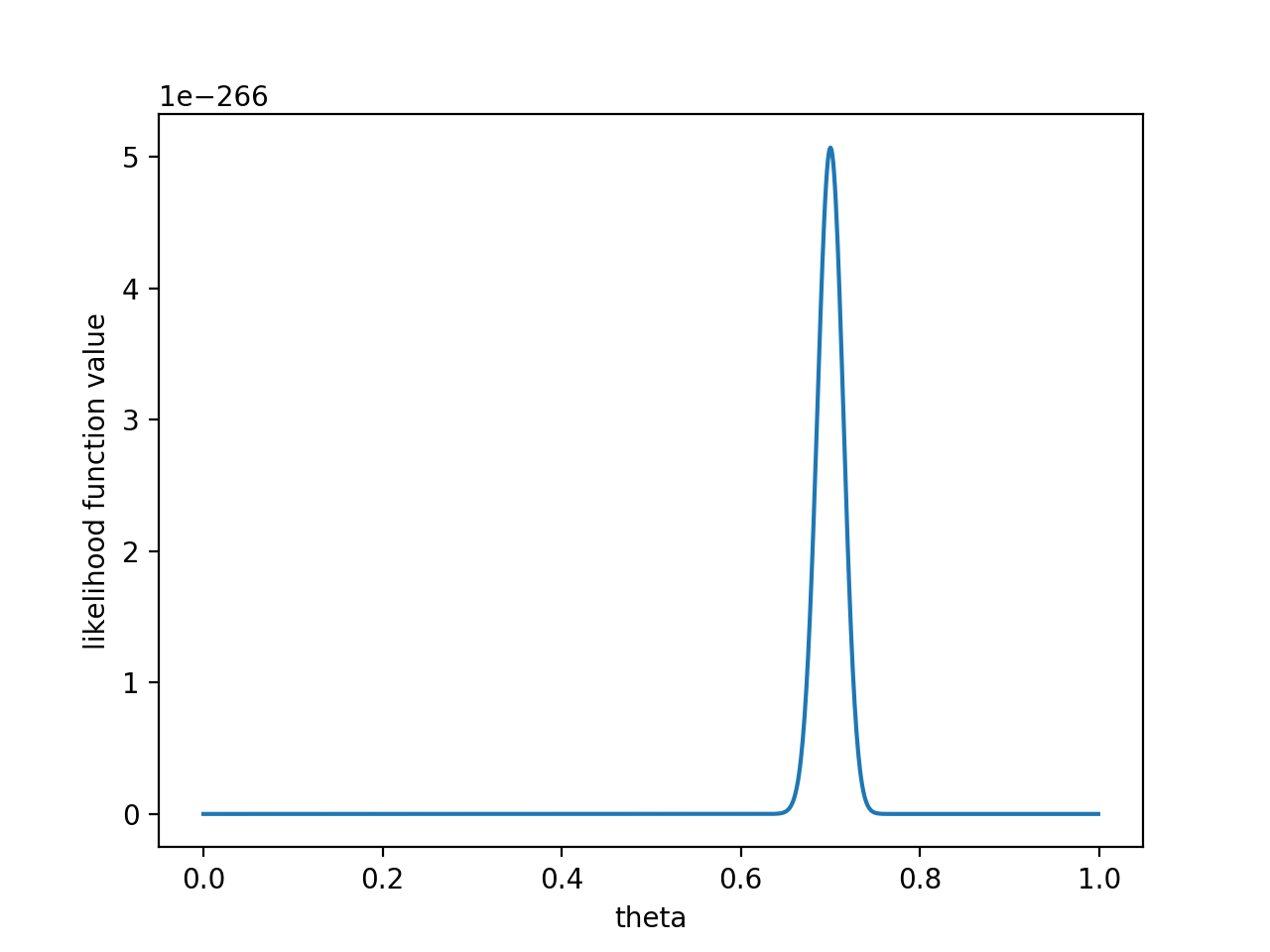如果仍然假设

P

(

θ

)

P(\theta)

为均值0.5，方差0.1的高斯函数，

P

(

x

0

∣

θ

)

P

(

θ

)

P(x_0 | \theta) P(\theta)

的函数图像为：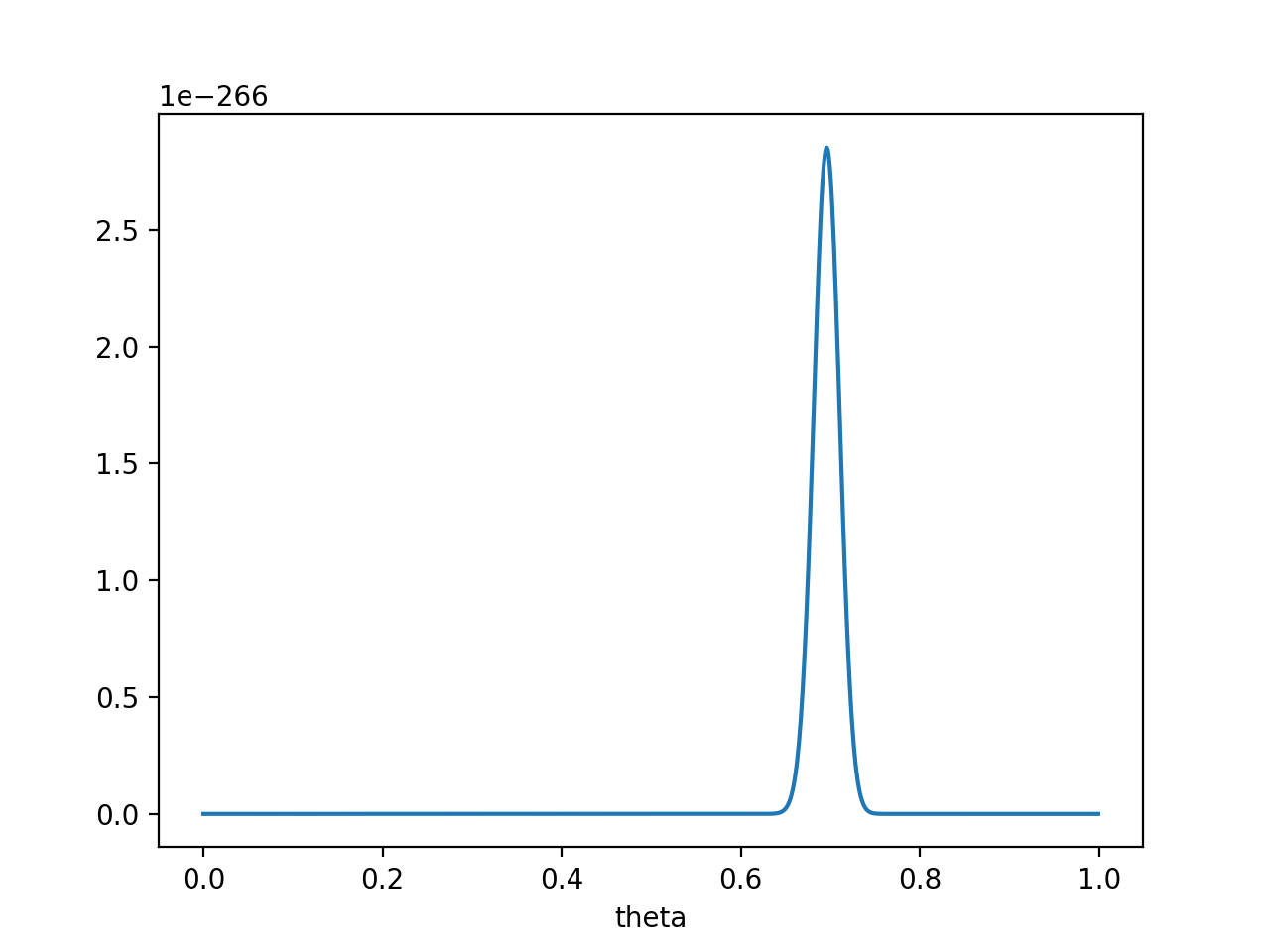在

θ

=

0.696

\theta = 0.696

处，

P

(

x

0

∣

θ

)

P

(

θ

)

P(x_0 | \theta) P(\theta)

取得最大值。
这样，就算一个考虑了先验概率的贝叶斯派，也不得不承认得把

θ

\theta

估计在0.7附近了。
PS. 要是遇上了顽固的贝叶斯派，认为

P

(

θ

=

0.5

)

=

1

P(\theta = 0.5) = 1

，那就没得玩了。。 无论怎么做实验，使用MAP估计出来都是

θ

=

0.5

\theta = 0.5

。这也说明，一个合理的先验概率假设是很重要的。（通常，先验概率能从数据中直接分析得到）
最大似然估计和最大后验概率估计的区别
相信读完上文，MLE和MAP的区别应该是很清楚的了。MAP就是多个作为因子的先验概率

P

(

θ

)

P(\theta)

。或者，也可以反过来，认为MLE是把先验概率

P

(

θ

)

P(\theta)

认为等于1，即认为

θ

\theta

是均匀分布。

如果有说错的或者没说清楚的地方，欢迎留言指教！如果您更好的见解，也欢迎留言交流！ 谢谢阅读！ 作者: nebulaf91
展开全文模式识别 机器学习 贝叶斯
• 本内容主要介绍概率论与统计学相关基础知识，主要涉及 联合概率、边缘概率和条件概率；贝叶斯公式、先验概率、验概率...最大似然估计、最大后验概率估计和贝叶斯估计 等。为机器学习中的贝叶斯分类提供相关基础知识。
  本内容主要介绍概率论与统计学相关基础知识，主要涉及 联合概率、边缘概率和条件概率；贝叶斯公式、先验概率、后验概率和似然函数；最大似然估计、最大后验概率估计和贝叶斯估计 等。为机器学习中的贝叶斯分类提供相关基础知识。
一、基础知识
1.1 概率与统计
概率论（Probability） 和 统计学（Statistics）看似两个相近的概念，其实研究的问题刚好相反。概率论 是集中研究概率及随机现象的数学分支，是研究随机性或不确定性等现象的数学。统计学 是在数据分析的基础上，研究如何测定、收集、整理、归纳和分析反映数据，以便给出正确消息的科学。
概率论是给定条件（已知模型和参数）下，对要发生的事件（新输入数据）的预测。统计推断是在给定数据（训练数据）下，对数据生成方式（模型和参数）的归纳总结。简单地说，概率论是已知模型和参数，推数据；统计学是已知数据，推模型和参数。概率论是统计学的数学基础，统计学是对概率论的应用。
1.2 联合概率、边缘概率和条件概率
1.2.1 联合概率
假设有随机变量

X

X

和

Y

Y

，此时，

P

(

X

=

a

,

Y

=

b

)

P(X=a,Y=b)

用于表示

X

=

a

X=a

且

Y

=

b

Y=b

的概率。这类包含多个条件且所有条件同时成立的概率称为 联合概率。我们也可以使用

P

(

a

,

b

)

P(a,b)

或

P

(

a

b

)

P(ab)

表示联合概率。
1.2.2 边缘概率
边缘概率是与联合概率对应的，

P

(

X

=

a

)

P(X=a)

或

P

(

Y

=

b

)

​

P(Y=b)​

，这类仅与单个随机变量有关的概率称为 边缘概率。
1.2.3 条件概率
条件概率 表示在条件

Y

=

b

Y=b

成立的情况下，

X

=

a

X=a

的概率，记作

P

(

X

=

a
&ThickSpace;

∣
&ThickSpace;

Y

=

b

)

P(X=a\;| \; Y=b)

。它具有如下性质：在条件

Y

=

b

Y=b

下

X

X

的条件分布，也是一种

X

X

的概率分布，因此穷举

X

X

的可取值之后，所有这些值对应的概率之和为 1，即

∑

a

P

(

X

=

a
&ThickSpace;

∣
&ThickSpace;

Y

=

b

)

=

1

\sum_a P(X=a \; | \; Y=b) = 1

。
1.2.4 联合概率与边缘概率的关系

(1)

P

(

X

=

a

)

=

∑

b

P

(

X

=

a

,

Y

=

b

)

P(X=a) = \sum_b P(X=a, Y=b) \tag{1}

(2)

P

(

Y

=

b

)

=

∑

a

P

(

X

=

a

,

Y

=

b

)

P(Y=b) = \sum_a P(X=a, Y=b) \tag{2}

1.2.5 联合概率、边缘概率与条件概率之间的关系

(3)

P

(

X

=

a

,

Y

=

b

)

=

P

(

X

=

a
&ThickSpace;

∣
&ThickSpace;

Y

=

b

)

P

(

Y

=

b

)

=

P

(

Y

=

b
&ThickSpace;

∣
&ThickSpace;

X

=

a

)

P

(

X

=

a

)

\begin{aligned} P(X=a,Y=b) &amp;= P(X=a \; | \; Y=b)P(Y=b) \\\\ &amp;= P(Y=b \; | \; X=a)P(X=a) \end{aligned} \tag{3}

(4)

P

(

X

=

a
&ThickSpace;

∣
&ThickSpace;

Y

=

b

)

=

P

(

X

=

a

,

Y

=

b

)

P

(

Y

=

b

)

P(X=a \; | \; Y=b) = \frac{P(X=a, Y=b)}{P(Y=b)} \tag{4}

1.3 全概率公式
假设随机变量

X

​

X​

的所有可能取值为

(

a

1

,

a

2

,

⋯
&ThinSpace;

,

a

n

)

​

(a_1,a_2,\cdots,a_n)​

，它们构成一个完备事件组，即它们两两互斥，其和为全集；并且

P

(

X

=

a

i

)

​

P(X=a_i)​

大于

0

​

0​

，则对任意事件

Y

=

b

​

Y=b​

有

(5)

P

(

Y

=

b

)

=

P

(

Y

=

b
&ThickSpace;

∣
&ThickSpace;

X

=

a

1

)

P

(

X

=

a

1

)

+

⋯

+

P

(

Y

=

b
&ThickSpace;

∣
&ThickSpace;

X

=

a

n

)

P

(

X

=

a

n

)

=

∑

i

=

1

n

P

(

Y

=

b
&ThickSpace;

∣
&ThickSpace;

X

=

a

i

)

P

(

X

=

a

i

)

\begin{aligned} P(Y=b) &amp;= P(Y=b \; | \; X=a_1)P(X=a_1) + \cdots + P(Y=b \; | \; X=a_n)P(X=a_n) \\\\ &amp;= \sum_{i=1}^{n} P(Y=b \; | \; X=a_i)P(X=a_i) \end{aligned} \tag{5}

上面的公式称为 全概率公式。它将对复杂事件

Y

=

b

​

Y=b​

的概率问题 转化为在不同情况下发生的简单事件的概率的求和问题 。
1.4 贝叶斯公式

(6)

P

(

Y

∣

X

)

=

P

(

X

∣

Y

)

P

(

Y

)

P

(

X

)

P(Y|X) = \frac{P(X|Y)P(Y)}{P(X)} \tag{6}

上面的公式称为 贝叶斯公式，也叫做 贝叶斯定理 或 贝叶斯法则。
1.5 先验概率、后验概率和似然函数
先验概率（Prior Probability）是指根据以往经验和分析得到的概率。
后验概率（Posterior Probability）是指在得到 “结果” 的信息后重新修正的概率。
在统计学中，似然函数（Likelihood Function）是一种关于统计模型参数的函数。给定输出

X

​

X​

时，关于参数

θ

​

\theta​

的似然函数

L

(

θ

∣

X

)

​

L(\theta|X)​

（在数值上）等于给定参数

θ

​

\theta​

后变量

X

​

X​

的概率：

L

(

θ

∣

X

)

=

P

(

X

∣

θ

)

​

L(\theta|X) = P(X|\theta)​

。
二、概率模型参数估计方法
假如我们现在有一组观测数据（即数据集，样本），并且我们知道它们是从某一种分布中随机取出来的，但是我们并不知道这个分布的具体参数，即 “模型已定，参数未知”。那我们应该如何去估计参数呢？
对于参数估计，统计学界的两个学派分别提供了不同的解决方案：频率学派 认为参数虽然未知，但却是客观存在的固定值，因此，可通过优化似然函数等准则来确定参数值；贝叶斯学派 则认为参数是未观察到的随机变量，其本身也可有分布，因此，可假定参数服从一个先验分布，然后基于观测到的数据来计算参数的后验分布。
下面将介绍三种概率模型参数估计方法，分别为 源自频率学派的最大似然估计，以及源自贝叶斯学派的最大后验概率估计和贝叶斯估计。
2.1 最大似然估计
最大似然估计（Maximum Likelihood Estimation，MLE，也叫极大似然估计）是建立在极大似然原理的基础上的一个统计方法，是概率论在统计学中的应用。其核心思想是：认为当前发生的事件（即观测数据）是概率最大的事件，则使得当前发生的事件发生概率最大的参数就是最好的参数。
在最大似然估计中，认为参数为固定的值，只是其值未知；然后基于观测数据，通过最大化似然函数来求解参数。
注意：对一个独立同分布的数据集来说，总体的似然就是每个样本似然的乘积。
2.1.1 示例一：二项分布的最大似然估计（抛硬币）
针对抛硬币的例子，抛 10 次的结果为：

0

，

0

，

0

，

1

，

0

，

0

，

0

，

1

，

0

，

0

​

0，0，0，1，0，0，0，1，0，0​

，，其中正面朝上为

1

​

1​

，反面朝上为

0

​

0​

，求这个硬币正面朝上的概率是多大？
我们知道每次抛硬币都是一次二项分布，设正面朝上的概率为

θ

​

\theta​

，那么似然函数为：

(7)

L

(

X

;

θ

)

=

∏

i

=

1

n

P

(

x

i

,

θ

)

=

∏

i

=

1

n

θ

x

i

(

1

−

θ

)

1

−

x

i

L(X; \theta) = \prod_{i=1}^{n} P(x_i, \theta) = \prod_{i=1}^{n}\theta^{x_i}(1-\theta)^{1-x_i} \tag{7}

其中，正面朝上时，

x

i

=

1

​

x_i =1​

；反面朝上时，

x

i

=

0

​

x_i=0​

。
为了求解方便，我们通常会将似然函数转成对数似然函数，然后再求解(可以转成对数似然函数的主要原因是对数函数并不影响函数的凹凸性。)。则有

(8)

ln

⁡

L

(

X

;

θ

)

=

ln

⁡

∏

i

=

1

n

θ

x

i

(

1

−

θ

)

1

−

x

i

=

∑

i

=

1

n

ln

⁡

(

θ

x

i

(

1

−

θ

)

1

−

x

i

)

=

∑

i

=

1

n

(

ln

⁡

θ

x

i

+

ln

⁡

(

1

−

θ

)

1

−

x

i

)

=

∑

i

=

1

n

(

x

i

ln

⁡

θ

+

(

1

−

x

i

)

ln

⁡

(

1

−

θ

)

)

\begin{aligned} \ln L(X; \theta) &amp;= \ln \prod_{i=1}^{n}\theta^{x_i}(1-\theta)^{1-x_i} \\ &amp;= \sum_{i=1}^{n} \ln (\theta^{x_i}(1-\theta)^{1-x_i}) \\ &amp;= \sum_{i=1}^{n} (\ln \theta^{x_i} + \ln (1-\theta)^{1-x_i}) \\ &amp;= \sum_{i=1}^{n} (x_i \ln \theta + (1-x_i)\ln (1-\theta)) \end{aligned} \tag{8}

对其求导，得

(9)

∂

∂

θ

ln

⁡

L

(

X

;

θ

)

=

∑

i

=

1

n

∂

∂

θ

(

x

i

ln

⁡

θ

+

(

1

−

x

i

)

ln

⁡

(

1

−

θ

)

)

=

∑

i

=

1

n

x

i

∂

∂

θ

ln

⁡

θ

+

∑

i

=

1

n

(

1

−

x

i

)

∂

∂

θ

ln

⁡

(

1

−

θ

)

=

1

θ

∑

i

=

1

n

x

i

−

1

1

−

θ

∑

i

=

1

n

(

1

−

x

i

)

\begin{aligned} \frac{\partial}{\partial \theta} \ln L(X; \theta) &amp;= \sum_{i=1}^{n} \frac{\partial}{\partial \theta}(x_i \ln \theta + (1-x_i)\ln (1-\theta)) \\ &amp;= \sum_{i=1}^{n} x_i \frac{\partial}{\partial \theta} \ln \theta + \sum_{i=1}^{n} (1-x_i) \frac{\partial}{\partial \theta} \ln (1-\theta) \\ &amp;= \frac{1}{\theta} \sum_{i=1}^{n} x_i - \frac{1}{1-\theta} \sum_{i=1}^{n} (1-x_i) \end{aligned} \tag{9}

令导数为

0

0

，可求得

(10)

θ

^

=

1

n

∑

i

=

1

n

x

i

\hat{\theta} = \frac{1}{n}\sum_{i=1}^{n} x_i \tag{10}

将上面的观测数据代入，可求得

θ

^

=

0.2

\hat{\theta} = 0.2

。
2.1.2 实例二：正态分布的最大似然估计
假设样本服从正态分布

N

(

μ

,

σ

2

)

N ~ (\mu, \sigma^2)

，则其似然函数为

(11)

L

(

μ

,

σ

2

)

=

∏

i

=

1

n

1

2

π

σ

e

−

(

x

i

−

μ

)

2

2

σ

2

L(\mu, \sigma^2) =\prod_{i=1}^{n} \frac{1}{\sqrt{2\pi}\sigma} e^{-\frac{(x_i - \mu)^2}{2\sigma^2}} \tag{11}

对其求对数得

(12)

ln

⁡

L

(

μ

,

σ

2

)

=

∑

i

=

1

n

ln

⁡

1

2

π

σ

e

−

(

x

i

−

μ

)

2

2

σ

2

=

∑

i

=

1

n

ln

⁡

1

2

π

σ

−

∑

i

=

1

n

(

x

i

−

μ

)

2

2

σ

2

=

∑

i

=

1

n

ln

⁡

1

2

π

+

∑

i

=

1

n

ln

⁡

1

σ

−

∑

i

=

1

n

(

x

i

−

μ

)

2

2

σ

2

=

n

ln

⁡

1

2

π

−

n

2

ln

⁡

σ

2

−

1

2

σ

2

∑

i

=

1

n

(

x

i

−

μ

)

2

\begin{aligned} \ln L(\mu, \sigma^2) &amp;= \sum_{i=1}^{n} \ln \frac{1}{\sqrt{2\pi}\sigma} e^{-\frac{(x_i - \mu)^2}{2\sigma^2}} \\ &amp;= \sum_{i=1}^{n} \ln \frac{1}{\sqrt{2\pi}\sigma} - \sum_{i=1}^{n} \frac{(x_i - \mu)^2}{2\sigma^2} \\ &amp;= \sum_{i=1}^{n} \ln \frac{1}{\sqrt{2\pi}} + \sum_{i=1}^{n} \ln \frac{1}{\sigma} - \sum_{i=1}^{n} \frac{(x_i - \mu)^2}{2\sigma^2} \\ &amp;= n \ln \frac{1}{\sqrt{2\pi}} - \frac{n}{2} \ln \sigma^2 - \frac{1}{2\sigma^2} \sum_{i=1}^{n} (x_i - \mu)^2 \end{aligned} \tag{12}

分别对

μ

\mu

，

σ

2

\sigma^2

求偏导，并令偏导数为 0，得

(13)

{

∂

∂

μ

ln

⁡

L

(

μ

,

σ

2

)

=

1

σ

2

∑

i

=

1

n

(

x

i

−

μ

)

=

1

σ

2

(

∑

i

=

1

n

x

i

−

n

μ

)

=

0

∂

∂

σ

2

ln

⁡

L

(

μ

,

σ

2

)

=

−

n

2

σ

2

+

1

2

σ

4

∑

i

=

1

n

(

x

i

−

μ

)

2

=

0

\left \{ \begin{array}{cc} \begin{aligned} &amp;\frac{\partial}{\partial \mu} \ln L(\mu, \sigma^2) =\frac{1}{\sigma^2} \sum_{i=1}^n(x_i - \mu) =\frac{1}{\sigma^2} (\sum_{i=1}^n x_i - n\mu) =0 \\\\ &amp;\frac{\partial}{\partial \sigma^2} \ln L(\mu, \sigma^2) =-\frac{n}{2\sigma^2} + \frac{1}{2\sigma^4}\sum_{i=1}^{n} (x_i - \mu)^2 =0 \end{aligned} \end{array} \right. \tag{13}

求得

(14)

{

μ

^

=

1

n

∑

i

=

1

n

x

i

=

x

ˉ

σ

2

^

=

1

n

∑

i

=

1

n

(

x

i

−

x

ˉ

)

2

\left \{ \begin{array}{cc} \begin{aligned} &amp;\hat{\mu} = \frac{1}{n} \sum_{i=1}^{n}x_i = \bar{x} \\\\ &amp;\hat{\sigma^2} = \frac{1}{n} \sum_{i=1}^{n} (x_i - \bar{x})^2 \end{aligned} \end{array} \right. \tag{14}

2.1.3 求解步骤
最大似然估计的求解步骤：
确定似然函数。  将似然函数转换为对数似然函数。  求导数，并令其为

0

0

。  求解得到模型中参数的最优值。
极大似然估计只关注当前的观测数据，也就是只关注当前发生的事件，不考虑事件的先验情况。由于计算简单，而且不需要关注先验知识，因此在机器学习中的应用非常广，最常见的就是 Logistic 回归。
2.2 最大后验概率估计
在最大似然估计中，是求使似然函数最大的参数。现在问题稍微复杂一点点，假如这个参数有一个先验概率呢？比如，上面的抛硬币的例子，我们的经验告诉我们，硬币一般都是匀称的，也就是

θ

=

0.5

\theta = 0.5

的概率最大，

θ

=

0.2

​

\theta = 0.2​

的概率比较小。这个时候我们应该怎么估计参数呢？这就是 最大后验概率估计（Maximum a Posteriori Estimation，MAP）要考虑的问题。
通过前面的介绍，我们了解到在最大似然估计中，认为参数为固定的值，只是其值未知，通过最大化似然函数来求解参数。但是在最大后验概率估计中，认为参数是一个随机变量，其本身也具有某种概率分布，称为先验分布。在求解时，除了要考虑似然函数

P

(

X

∣

θ

)

​

P(X|\theta)​

之外，还要考虑

θ

​

\theta​

的先验分布

P

(

θ

)

​

P(\theta)​

，因此其认为是

P

(

X

∣

θ

)

P

(

θ

)

​

P(X|\theta) P(\theta)​

取最大值的

θ

​

\theta​

就是最好的

θ

​

\theta​

。此时要最大化的函数变为

P

(

X

∣

θ

)

P

(

θ

)

​

P(X|\theta) P(\theta)​

，由于

X

​

X​

的先验分布

P

(

X

)

​

P(X)​

是固定的，因此最大化函数可变为

P

(

X

∣

θ

)

P

(

θ

)

/

P

(

X

)

​

{P(X|\theta)P(\theta)} / {P(X)}​

，根据贝叶斯公式可知，实际上要最大化的函数是

P

(

θ

∣

X

)

​

P(\theta|X)​

，其为

θ

​

\theta​

的后验概率。最大化后验概率估计可以看作是正则化的最大似然估计，当然机器学习或深度学习中的正则化通常是加法，而在最大后验概率估计中采用的是乘法，

P

(

θ

)

​

P(\theta)​

是正则项。在最大似然估计中，由于认为

θ

​

\theta​

是固定的，因此

P

(

θ

)

=

1

​

P(\theta) = 1​

。
2.2.1 示例一：参数

θ

\theta

的先验分布为 Beta 分布
我们继续使用上面的抛硬币的例子，假设参数

θ

​

\theta​

的先验分布为 Beta 分布。则

(15)

P

(

X

∣

θ

)

P

(

θ

)

=

∏

i

=

1

n

θ

x

i

(

1

−

θ

)

1

−

x

i

1

B

(

α

,

β

)

θ

α

−

1

(

1

−

θ

)

β

−

1

P(X|\theta)P(\theta) = \prod_{i=1}^{n}\theta^{x_i}(1-\theta)^{1-x_i} \frac{1}{B(\alpha, \beta)} \theta^{\alpha-1} (1-\theta)^{\beta-1} \tag{15}

对其求对数得

(16)

ln

⁡

P

(

X

∣

θ

)

P

(

θ

)

=

∑

i

=

1

n

ln

⁡

(

θ

x

i

(

1

−

θ

)

1

−

x

i

)

+

ln

⁡

1

B

(

α

,

β

)

+

ln

⁡

θ

α

−

1

+

ln

⁡

(

1

−

θ

)

β

−

1

=

ln

⁡

θ

∑

i

=

1

n

x

i

+

ln

⁡

(

1

−

θ

)

∑

i

=

1

n

(

1

−

x

i

)

−

ln

⁡

B

(

α

,

β

)

+

(

α

−

1

)

ln

⁡

θ

+

(

β

−

1

)

ln

⁡

(

1

−

θ

)

\begin{aligned} \ln P(X|\theta)P(\theta) &amp;= \sum_{i=1}^{n} \ln \left(\theta^{x_i}(1-\theta)^{1-x_i}\right) + \ln \frac{1}{B(\alpha, \beta)} + \ln \theta^{\alpha-1} + \ln (1-\theta)^{\beta-1} \\\\ &amp;= \ln \theta \sum_{i=1}^{n} x_i +\ln(1-\theta)\sum_{i=1}^{n} (1-x_i) \\ &amp;\quad\quad-\ln B(\alpha, \beta) +(\alpha-1) \ln \theta +(\beta - 1) \ln (1-\theta) \end{aligned} \tag{16}

对参数求导，得

(17)

∂

∂

θ

ln

⁡

P

(

X

∣

θ

)

P

(

θ

)

=

1

θ

∑

i

=

1

n

x

i

−

1

1

−

θ

∑

i

=

1

n

(

1

−

x

i

)

+

α

−

1

θ

−

β

−

1

1

−

θ

\begin{aligned} \frac{\partial}{\partial \theta} \ln P(X|\theta)P(\theta) &amp;= \frac{1}{\theta} \sum_{i=1}^{n} x_i -\frac{1}{1-\theta}\sum_{i=1}^{n}(1-x_i) +\frac{\alpha-1}{\theta} -\frac{\beta-1}{1-\theta} \end{aligned} \tag{17}

令其为

0

0

，求得

(18)

θ

^

=

∑

i

=

1

n

x

i

+

α

−

1

n

+

α

+

β

−

2

\hat{\theta} = \frac{\sum_{i=1}^{n}x_i + \alpha-1}{n+\alpha + \beta - 2} \tag{18}

假设 Beta 分布的参数分别为

α

=

3

\alpha=3

，

β

=

3

\beta = 3

，将上面的观测数据代入，可求得

(19)

θ

^

=

2

+

3

−

1

10

+

3

+

3

−

2

=

4

14

=

0.2857

\hat{\theta} = \frac{2 + 3 - 1}{10 + 3 + 3 - 2} = \frac{4}{14} = 0.2857 \tag{19}

2.2.2 求解步骤
最大后验概率估计的求解步骤：
确定参数的先验分布以及似然函数。确定参数的后验分布函数。将后验分布函数转换为对数函数。求对数函数的最大值（求导，解方程）
最大后验概率估计不只是关注当前的观测数据，还关注已经发生过的先验知识。
最大后验估计和最大似然估计的区别：最大后验估计允许我们把先验知识加入到估计模型中，这在样本很少的时候是很有用的（因此朴素贝叶斯在较少的样本下就能有很好的表现），因为样本很少的时候我们的观测结果很可能出现偏差，此时先验知识会把估计的结果“拉”向先验，实际的预估结果将会在先验结果的两侧形成一个顶峰。通过调节先验分布的参数，比如beta分布的α，β，我们还可以调节把估计的结果“拉”向先验的幅度，α，β越大，这个顶峰越尖锐。这样的参数，我们叫做预估模型的“超参数”。
2.3 贝叶斯估计
贝叶斯估计是最大后验概率估计的进一步扩展，贝叶斯估计同样假定参数是一个随机变量，但贝叶斯估计并不是直接估计出参数的某个特定值，而是估计参数的分布，这是贝叶斯估计与最大后验概率估计不同的地方。在贝叶斯估计中，先验分布是不可忽略的。
待添加……
参考：
 周志华《机器学习》  李航《统计学习方法》  贝叶斯估计、最大似然估计、最大后验概率估计  最大似然估计、最大后验估计、贝叶斯估计的对比  最大似然估计 （MLE） 最大后验概率（MAP）  极大似然估计详解
展开全文最大似然估计 贝叶斯估计 贝叶斯定理
• 贝叶斯估计、最大似然估计(MLE)、最大后验概率估计(MAP)这几个概念在机器学习和深度学习中经常碰到，读文章的时候还感觉挺明白，但独立思考时经常会傻傻分不清楚(��)，因此希望通过本文对其进行总结。 2. ...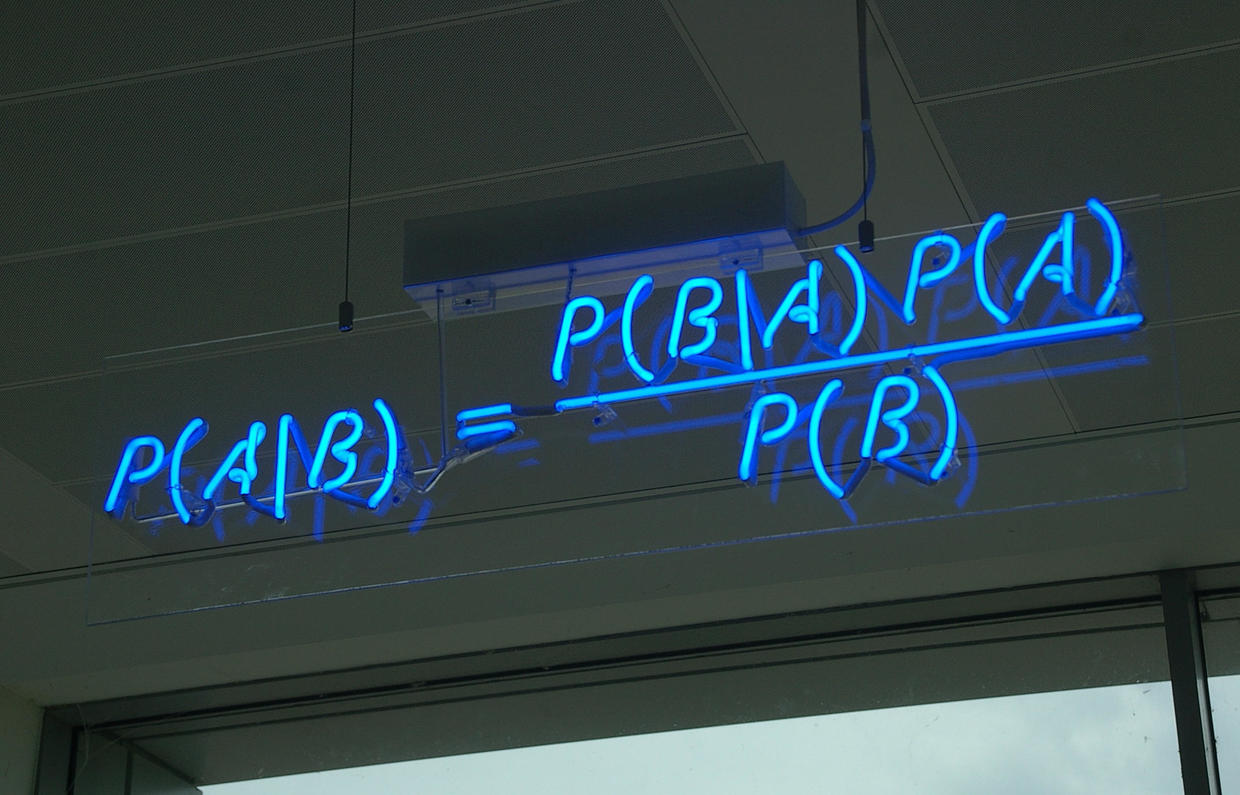文章作者：Tyan 博客：noahsnail.com  |  CSDN  |  简书
1. 引言
贝叶斯估计、最大似然估计(MLE)、最大后验概率估计(MAP)这几个概念在机器学习和深度学习中经常碰到，读文章的时候还感觉挺明白，但独立思考时经常会傻傻分不清楚(?)，因此希望通过本文对其进行总结。

2. 背景知识
**注：**由于概率与数理统计需要了解的背景知识很多，因此这里只列出了部分内容，且写的较简略，许多概念的学习需要根据标题自己查找答案。
2.1 概率与统计
概率统计是很多人都学过的内容，但概率论与统计学的关系是什么？先看一下概率论与统计学在维基百科中的定义：
概率论是集中研究概率及随机现象的数学分支，是研究随机性或不确定性等现象的数学。
统计学是在数据分析的基础上，研究如何测定、收集、整理、归纳和分析反映数据数据，以便给出正确消息的科学。

下面的一段话引自LarrB Wasserman的《All of Statistics》，对概率和统计推断的研究内容进行了描述：
The basic problem that we studB in probabilitB is:
Given a data generating process, what are the properities of the outcomes?

The basic problem of statistical inference is the inverse of probabilitB:
Given the outcomes, what can we saB about the process that generated the data?

概率论是在给定条件（已知模型和参数）下，对要发生的事件（新输入数据）的预测。统计推断是在给定数据（训练数据）下，对数据生成方式（模型和参数）的归纳总结。概率论是统计学的数学基础，统计学是对概率论的应用。
2.2 描述统计和推断统计
统计学分为描述统计学和推断统计学。描述统计，是统计学中描绘或总结观察量基本情况的统计总称。推断统计指统计学中研究如何根据样本数据去推断总体数量特征的方法。
描述统计是对数据的一种概括。描述统计是罗列所有数据，然后选择一些特征量（例如均值、方差、中位数、四分中位数等）对总体数据进行描述。推断统计是一种对数据的推测。推断统计无法获取所有数据，只能得到部分数据，然后根据得到的数据推测总体数据的情况。
2.3 联合概率和边缘概率
假设有随机变量

A

A

和

B

B

，此时

P

(

A

=

a

,

B

=

b

)

P(A=a,B=b)

用于表示

A

=

a

A=a

且

B

=

b

B=b

同时发生的概率。这类包含多个条件且所有条件同时成立的概率称为联合概率。请注意，联合概率并不是其中某个条件成立的概率，而是所有条件同时成立的概率。与之对应地，

P

(

A

=

a

)

P(A=a)

或

P

(

B

=

b

)

P(B=b)

这类仅与单个随机变量有关的概率称为边缘概率。
联合概率与边缘概率的关系如下：

P

(

A

=

a

)

=

∑

b

P

(

A

=

a

,

B

=

b

)

P(A=a)=\sum_{b}P(A=a,B=b)

P

(

B

=

b

)

=

∑

a

P

(

A

=

a

,

B

=

b

)

P(B=b)=\sum_{a}P(A=a,B=b)

2.4 条件概率
条件概率表示在条件

B

=

b

B=b

成立的情况下，

A

=

a

A=a

的概率，记作

P

(

A

=

a

∣

B

=

b

)

P(A=a|B=b)

，或者说条件概率是指事件

A

=

a

A=a

在另外一个事件

B

=

b

B=b

已经发生条件下的发生概率。为了简洁表示，后面省略a，b。
联合概率、边缘概率、条件概率的关系如下：

P

(

A

∣

B

)

=

P

(

A

,

B

)

P

(

B

)

P(A|B)=\frac {P(A,B)} {P(B)}

转换为乘法形式：

P

(

A

,

B

)

=

P

(

B

)

∗

P

(

A

∣

B

)

=

P

(

A

)

∗

P

(

B

∣

A

)

P(A,B)=P(B)*P(A|B)=P(A)*P(B|A)

2.5 全概率公式
如果事件

A

1

，

A

2

，

A

3

，

…

，

A

n

A_1，A_2，A_3，\ldots，A_n

构成一个完备事件组，即它们两两互不相容（互斥），其和为全集；并且

P

(

A

i

)

P(A_i)

大于0，则对任意事件

B

B

有

P

(

B

)

=

P

(

B

∣

A

1

)

P

(

A

1

)

+

P

(

B

∣

A

2

)

P

(

A

2

)

+

…

+

P

(

B

∣

A

n

)

P

(

A

n

)

=

∑

i

=

1

n

P

(

B

∣

A

i

)

P

(

A

i

)

P(B)=P(B|A_1)P(A_1)+P(B|A_2)P(A_2)+\ldots+ P(B|A_n)P(A_n)=\sum^n_{i=1}P(B|A_i)P(A_i)

上面的公式称为全概率公式。全概率公式是对复杂事件

A

A

的概率求解问题转化为了在不同情况下发生的简单事件的概率的求和问题。
2.6 贝叶斯公式
由条件概率的乘法形式可得：

P

(

A

∣

B

)

=

P

(

B

∣

A

)

P

(

B

)

∗

P

(

A

)

P(A|B)=\frac {P(B|A)} {P(B)}*P(A)

上面的式子称为贝叶斯公式，也叫做贝叶斯定理或贝叶斯法则。在贝叶斯定理中，每个名词都有约定俗成的名称：

P

(

A

∣

B

)

P(A|B)

是已知

B

B

发生后

A

A

的条件概率，也由于得自

B

B

的取值而被称作

A

A

的后验概率，表示事件

B

B

发生后，事件

A

A

发生的置信度。

P

(

A

)

P(A)

是

A

A

的先验概率或边缘概率，表示事件

A

A

发生的置信度。

P

(

B

∣

A

)

P(B|A)

是已知

A

A

发生后

B

B

的条件概率，也由于得自

A

A

的取值而被称作

B

B

的后验概率，也被称作似然函数。

P

(

B

)

P(B)

是

B

B

的先验概率或边缘概率，称为标准化常量。

P

(

B

∣

A

)

P

(

B

)

\frac {P(B|A)} {P(B)}

称为标准似然比(这个叫法很多，没找到标准统一的叫法)，表示事件

B

B

为事件

A

A

发生提供的支持程度。
因此贝叶斯公式可表示为：后验概率=似然函数先验概率/标准化常量=标准似然比先验概率。根据标准似然比的大小，可分为下面三种情况：
如果标准似然比

>

1

>1

，则先验概率

P

(

A

)

P(A)

得到增强，事件

B

B

的发生会增大事件

A

A

发生的可能性；如果标准似然比

=

1

=1

，则先验概率

P

(

A

)

P(A)

保持不变，事件

B

B

的发生不影响事件

A

A

发生的可能性；如果标准似然比

<

1

<1

，则先验概率

P

(

A

)

P(A)

得到削弱，事件

B

B

的发生会降低事件

A

A

发生的可能性。
由全概率公式、贝叶斯法则可得：

P

(

A

i

∣

B

)

=

P

(

B

∣

A

i

)

P

(

A

i

)

P

(

B

)

=

P

(

B

∣

A

i

)

P

(

A

i

)

∑

i

=

1

n

P

(

B

∣

A

i

)

P

(

A

i

)

P(A_i|B)=\frac {P(B|A_i)P(A_i)} {P(B)}=\frac {P(B|A_i)P(A_i)} {\sum^n_{i=1}P(B|A_i)P(A_i)}

2.7 似然与概率
在英文中，似然（likelihood）和概率（probability）是同义词，都指事件发生的可能性。但在统计中，似然与概率是不同的东西。概率是已知参数，对结果可能性的预测。似然是已知结果，对参数是某个值的可能性预测。
2.8 似然函数与概率函数
对于函数

P

(

x

∣

θ

)

P(x|\theta)

，从不同的观测角度来看可以分为以下两种情况：
如果

θ

\theta

已知且保持不变，

x

x

是变量，则

P

(

x

∣

θ

)

P(x|\theta)

称为概率函数，表示不同

x

x

出现的概率。如果

x

x

已知且保持不变，

θ

\theta

是变量，则

P

(

x

∣

θ

)

P(x|\theta)

称为似然函数，表示不同

θ

\theta

下，

x

x

出现的概率，也记作

L

(

θ

∣

x

)

L(\theta|x)

或

L

(

x

;

θ

)

L(x;\theta)

或

f

(

x

;

θ

)

f(x;\theta)

。
**注：**注意似然函数的不同写法。
2.9 推断统计中需要了解的一些概念
假设实际观测值与真实分布相关，试图根据观测值来推测真实分布由于观测值取值随机，因此由它们计算得到的估计值也是随机值估计方式多种多样，且不同估计方式得到的估计值也有所不同
样本、样本容量、参数统计、非参数统计、估计量、真实分布、经验分布。
2.10 频率学派与贝叶斯学派
**注：**频率学派与贝叶斯学派只是解决问题的角度不同。
频率学派与贝叶斯学派探讨「不确定性」这件事时的出发点与立足点不同。频率学派从「自然」角度出发，试图直接为「事件」本身建模，即事件

A

A

在独立重复试验中发生的频率趋于极限

p

p

，那么这个极限就是该事件的概率。
贝叶斯学派并不从试图刻画「事件」本身，而从「观察者」角度出发。贝叶斯学派并不试图说「事件本身是随机的」，或者「世界的本体带有某种随机性」，这套理论根本不言说关于「世界本体」的东西，而只是从「观察者知识不完备」这一出发点开始，构造一套在贝叶斯概率论的框架下可以对不确定知识做出推断的方法。
频率学派的代表是最大似然估计；贝叶斯学派的代表是最大后验概率估计。
2.11 共轭先验
在贝叶斯统计中，如果后验分布与先验分布属于同类，则先验分布与后验分布被称为共轭分布，而先验分布被称为似然函数的共轭先验。
2.12 Beta分布
在概率论中，Beta分布也称Β分布，是指一组定义在

(

0

,

1

)

(0,1)

区间的连续概率分布，有两个参数

α

,

β

>

0

\alpha,\beta>0

。Beta分布的概率密度为：
KaTeX parse error: No such environment: align at position 7: \begin{̲a̲l̲i̲g̲n̲}̲f(x;\alpha,\bet…其中，

Γ

(

z

)

\Gamma(z)

是

Γ

\Gamma

函数。随机变量

X

X

服从Beta分布写作

X

∼

B

e

t

a

(

α

,

β

)

X\sim Beta(\alpha,\beta)

。

3. 问题定义
以抛硬币为例，假设我们有一枚硬币，现在要估计其正面朝上的概率

θ

\theta

。为了对

θ

\theta

进行估计，我们进行了10次实验（独立同分布，i.i.d.），这组实验记为

X

=

x

1

，

x

2

，

…

，

x

10

X=x_1，x_2，\ldots，x_{10}

，其中正面朝上的次数为6次，反面朝上的次数为4次，结果为

(

1

,

0

,

1

,

1

,

0

,

0

,

0

,

1

,

1

,

1

)

(1,0,1,1,0,0,0,1,1,1)

。
4. 最大似然估计(MLE)
最大似然估计，英文为Maximum Likelihood Estimation，简写为MLE，也叫极大似然估计，是用来估计概率模型参数的一种方法。最大似然估计的思想是使得观测数据（样本）发生概率最大的参数就是最好的参数。
对一个独立同分布的样本集来说，总体的似然就是每个样本似然的乘积。针对抛硬币的问题，似然函数可写作：

L

(

X

;

θ

)

=

∏

i

=

0

n

P

(

x

i

∣

θ

)

=

θ

6

(

1

−

θ

)

4

L(X;\theta)=\prod_{i=0}^nP(x_i|\theta)=\theta^6(1-\theta)^4

根据最大似然估计，使

L

(

X

;

θ

)

L(X;\theta)

取得最大值的

θ

\theta

即为估计结果，令

L

(

X

;

θ

)

′

=

0

L(X;\theta)\prime =0

可得

θ

^

=

0.6

\hat{\theta}=0.6

。似然函数图如下：由于总体的似然就是每个样本似然的乘积，为了求解方便，我们通常会将似然函数转成对数似然函数，然后再求解。可以转成对数似然函数的主要原因是对数函数并不影响函数的凹凸性。因此上式可变为：

l

n

L

(

X

;

θ

)

=

l

n

∏

i

=

0

n

P

(

x

i

∣

θ

)

=

∑

i

=

0

n

l

n

(

P

(

x

i

∣

θ

)

)

=

6

l

n

(

θ

)

+

4

l

n

(

1

−

θ

)

lnL(X;\theta)=ln\prod_{i=0}^nP(x_i|\theta)=\sum_{i=0}^nln(P(x_i|\theta))=6ln(\theta)+4ln(1-\theta)

令

l

n

(

L

(

X

;

θ

)

′

)

=

0

ln(L(X;\theta)\prime) =0

可得

θ

^

=

0.6

\hat{\theta}=0.6

。
正态分布的最大似然估计
假设样本服从正态分布

N

∼

(

μ

,

σ

2

)

N\sim(\mu,\sigma^2)

，则其似然函数为

L

(

μ

,

σ

2

)

=

∏

i

=

0

n

1

2

π

σ

e

−

(

x

i

−

μ

)

2

2

σ

2

L(\mu,\sigma^2)=\prod_{i=0}^n \frac {1} {\sqrt{2\pi} \sigma}e^{-\frac {(x_i-\mu)^2} {2\sigma^2}}

对其取对数得：

l

n

L

(

μ

,

σ

2

)

=

−

n

2

l

n

(

2

π

)

−

n

2

l

n

(

σ

2

)

−

1

2

σ

2

∑

i

=

0

n

(

x

i

−

μ

)

2

lnL(\mu,\sigma^2)=-\frac {n} {2}ln(2\pi) - \frac {n} {2} ln(\sigma^2) - \frac {1} {2\sigma^2} \sum_{i=0}^n(x_i-\mu)^2

分别对

μ

，

σ

2

\mu，\sigma^2

求偏导，并令偏导数为0，得：

{

∂

l

n

L

(

μ

,

σ

2

)

∂

μ

=

1

σ

2

∑

i

=

0

n

(

x

i

−

μ

)

=

0

∂

l

n

L

(

μ

,

σ

2

)

∂

σ

2

=

−

n

2

σ

2

+

1

2

σ

4

∑

i

=

0

n

(

x

i

−

μ

)

2

=

0

\begin{cases} \frac {\partial lnL(\mu,\sigma^2)} {\partial \mu}= \frac {1} {\sigma^2} \sum_{i=0}^n(x_i-\mu) =0\\\\ \frac {\partial lnL(\mu,\sigma^2)} {\partial \sigma^2}= -\frac {n} {2\sigma^2} + \frac {1} {2\sigma^4}\sum_{i=0}^n(x_i-\mu)^2 =0 \end{cases}

解得：

{

μ

^

=

1

n

∑

i

=

0

n

x

i

=

x

ˉ

σ

2

^

=

1

n

∑

i

=

0

n

(

x

i

−

x

ˉ

)

2

\begin{cases} \hat{\mu}= \frac {1} {n} \sum_{i=0}^nx_i=\bar{x}\\\\ \hat{\sigma^2} = \frac {1} {n} \sum_{i=0}^n(x_i-\bar{x})^2 \end{cases}

μ

^

，

σ

2

^

\hat{\mu}，\hat{\sigma^2}

就是正态分布中

μ

，

σ

2

\mu，\sigma^2

的最大似然估计。
最大似然估计的求解步骤：
确定似然函数将似然函数转换为对数似然函数求对数似然函数的最大值（求导，解似然方程）

5. 最大后验概率估计(MAP)
最大后验概率估计，英文为Maximum A Posteriori Estimation，简写为MAP。回到抛硬币的问题，最大似然估计认为使似然函数

P

(

X

∣

θ

)

P(X|\theta)

最大的参数

θ

\theta

即为最好的

θ

\theta

，此时最大似然估计是将

θ

\theta

看作固定的值，只是其值未知；最大后验概率分布认为

θ

\theta

是一个随机变量，即

θ

\theta

具有某种概率分布，称为先验分布，求解时除了要考虑似然函数

P

(

X

∣

θ

)

P(X|\theta)

之外，还要考虑

θ

\theta

的先验分布

P

(

θ

)

P(\theta)

，因此其认为使

P

(

X

∣

θ

)

P

(

θ

)

P(X|\theta)P(\theta)

取最大值的

θ

\theta

就是最好的

θ

\theta

。此时要最大化的函数变为

P

(

X

∣

θ

)

P

(

θ

)

P(X|\theta)P(\theta)

，由于

X

X

的先验分布

P

(

X

)

P(X)

是固定的（可通过分析数据获得，其实我们也不关心

X

X

的分布，我们关心的是

θ

\theta

），因此最大化函数可变为

P

(

X

∣

θ

)

P

(

θ

)

P

(

X

)

\frac {P(X|\theta)P(\theta)} {P(X)}

，根据贝叶斯法则，要最大化的函数

P

(

X

∣

θ

)

P

(

θ

)

P

(

X

)

=

P

(

θ

∣

X

)

\frac {P(X|\theta)P(\theta)} {P(X)}=P(\theta|X)

，因此要最大化的函数是

P

(

θ

∣

X

)

P(\theta|X)

，而

P

(

θ

∣

X

)

P(\theta|X)

是

θ

\theta

的后验概率。最大后验概率估计可以看作是正则化的最大似然估计，当然机器学习或深度学习中的正则项通常是加法，而在最大后验概率估计中采用的是乘法，

P

(

θ

)

P(\theta)

是正则项。在最大似然估计中，由于认为

θ

\theta

是固定的，因此

P

(

θ

)

=

1

P(\theta)=1

。
最大后验概率估计的公式表示：

a

r

g

m

a

x

θ

P

(

θ

∣

X

)

=

a

r

g

m

a

x

θ

P

(

X

∣

θ

)

P

(

θ

)

P

(

X

)

∝

a

r

g

m

a

x

θ

P

(

X

∣

θ

)

P

(

θ

)

\mathop{argmax}_{\theta}P(\theta|X)=\mathop{argmax}_{\theta}\frac {P(X|\theta)P(\theta)} {P(X)}\propto \mathop{argmax}_{\theta}P(X|\theta)P(\theta)

在抛硬币的例子中，通常认为

θ

=

0.5

\theta=0.5

的可能性最大，因此我们用均值为

0.5

0.5

，方差为

0.1

0.1

的高斯分布来描述

θ

\theta

的先验分布，当然也可以使用其它的分布来描述

θ

\theta

的先验分布。

θ

\theta

的先验分布为：

1

2

π

σ

e

−

(

θ

−

μ

)

2

2

σ

2

=

1

10

2

π

e

−

50

(

θ

−

0.5

)

2

\frac {1} {\sqrt{2\pi}\sigma}e^{-\frac {(\theta-\mu)^2} {2\sigma^2}} = \frac {1} {10\sqrt{2\pi}}e^{-50(\theta-0.5)^2}

先验分布的函数图如下：在最大似然估计中，已知似然函数为

P

(

X

∣

θ

)

=

θ

6

(

1

−

θ

)

4

P(X|\theta)=\theta^6(1-\theta)^4

，因此：

P

(

X

∣

θ

)

P

(

θ

)

=

θ

6

×

(

1

−

θ

)

4

×

1

10

2

π

×

e

−

50

(

θ

−

0.5

)

2

P(X|\theta)P(\theta)=\theta^6\times (1-\theta)^4\times \frac {1} {10\sqrt{2\pi}}\times e^{-50(\theta-0.5)^2}

转换为对数函数：

l

n

(

P

(

X

∣

θ

)

P

(

θ

)

)

=

l

n

(

θ

6

×

(

1

−

θ

)

4

×

1

10

2

π

×

e

−

50

(

θ

−

0.5

)

2

)

=

6

l

n

(

θ

)

+

4

l

n

(

1

−

θ

)

+

l

n

(

1

10

2

π

)

−

50

(

θ

−

0.5

)

2

ln(P(X|\theta)P(\theta))=ln(\theta^6\times (1-\theta)^4 \times \frac {1} {10\sqrt{2\pi}}\times e^{-50(\theta-0.5)^2})=6ln(\theta)+4ln(1-\theta)+ln(\frac {1} {10\sqrt{2\pi}})-50(\theta-0.5)^2

令

l

n

(

P

(

X

∣

θ

)

P

(

θ

)

)

′

=

0

ln(P(X|\theta)P(\theta))\prime=0

，可得：

100

θ

3

−

150

θ

2

+

40

θ

+

6

=

0

100\theta^3-150\theta^2+40\theta+6=0

由于

0

≤

θ

≤

1

0\le\theta\le1

，解得：

θ

^

≈

0.529

\hat{\theta}\approx0.529

。

P

(

X

∣

θ

)

P

(

θ

)

P(X|\theta)P(\theta)

的函数图像如下，基本符合

θ

\theta

的估计值

θ

^

\hat{\theta}

：如果我们用均值为

0.6

0.6

，方差为

0.1

0.1

的高斯分布来描述

θ

\theta

的先验分布，则

θ

^

=

0.6

\hat{\theta}=0.6

。由此可见，在最大后验概率估计中，

θ

\theta

的估计值与

θ

\theta

的先验分布有很大的关系。这也说明一个合理的先验概率假设是非常重要的。如果先验分布假设错误，则会导致估计的参数值偏离实际的参数值。
先验分布为Beta分布
如果用

α

=

3

,

β

=

3

\alpha=3,\beta=3

的Beta分布来描述

θ

\theta

的先验分布，则

P

(

X

∣

θ

)

P

(

θ

)

=

θ

6

×

(

1

−

θ

)

4

×

1

B

(

α

,

β

)

×

θ

α

−

1

(

1

−

θ

)

β

−

1

P(X|\theta)P(\theta)=\theta^6\times (1-\theta)^4\times \frac {1} {B(\alpha,\beta)}\times \theta^{\alpha-1}(1-\theta)^{\beta-1}

令

P

(

X

∣

θ

)

P

(

θ

)

′

=

0

P(X|\theta)P(\theta)\prime=0

求解可得：

θ

^

=

α

+

5

α

+

β

+

8

=

8

3

+

3

+

8

≈

0.57

\hat{\theta}=\frac {\alpha+5} {\alpha + \beta +8}=\frac {8} {3 + 3 +8}\approx 0.57

B

e

t

a

(

3

,

3

)

Beta(3,3)

的概率密度图像如下图：最大后验概率估计的求解步骤：
确定参数的先验分布以及似然函数确定参数的后验分布函数将后验分布函数转换为对数函数求对数函数的最大值（求导，解方程）

6. 贝叶斯估计
贝叶斯估计是最大后验估计的进一步扩展，贝叶斯估计同样假定

θ

\theta

是一个随机变量，但贝叶斯估计并不是直接估计出

θ

\theta

的某个特定值，而是估计

θ

\theta

的分布，这是贝叶斯估计与最大后验概率估计不同的地方。在贝叶斯估计中，先验分布

P

(

X

)

P(X)

是不可忽略的。回到抛硬币的例子中，在已知

X

X

的情况下，描述

θ

\theta

的分布即描述

P

(

θ

∣

X

)

P(\theta|X)

，

P

(

θ

∣

X

)

P(\theta|X)

是一种后验分布。如果后验分布的范围较窄，则估计值的准确度相对较高，反之，如果后验分布的范围较广，则估计值的准确度就较低。
贝叶斯公式：

P

(

θ

∣

X

)

=

P

(

X

∣

θ

)

P

(

θ

)

P

(

X

)

P(\theta|X)=\frac {P(X|\theta)P(\theta)} {P(X)}

在连续型随机变量中，由于

P

(

X

)

=

∫

Θ

P

(

X

∣

θ

)

P

(

θ

)

d

θ

P(X)=\int_{\Theta}P(X|\theta)P(\theta)d\theta

，因此贝叶斯公式变为：

P

(

θ

∣

X

)

=

P

(

X

∣

θ

)

P

(

θ

)

∫

Θ

P

(

X

∣

θ

)

P

(

θ

)

d

θ

P(\theta|X)=\frac {P(X|\theta)P(\theta)} {\int_{\Theta}P(X|\theta)P(\theta)d\theta}

从上面的公式中可以看出，贝叶斯估计的求解非常复杂，因此选择合适的先验分布就非常重要。一般来说，计算积分

∫

θ

P

(

X

∣

θ

)

P

(

θ

)

d

θ

\int_{\theta}P(X|\theta)P(\theta)d\theta

是不可能的。对于这个抛硬币的例子来说，如果使用共轭先验分布，就可以更好的解决这个问题。二项分布参数的共轭先验是Beta分布，由于

θ

\theta

的似然函数服从二项分布，因此在贝叶斯估计中，假设

θ

\theta

的先验分布服从

P

(

θ

)

∼

B

e

t

a

(

α

,

β

)

P(\theta)\sim Beta(\alpha, \beta)

，Beta分布的概率密度公式为：

f

(

x

;

α

,

β

)

=

1

B

(

α

,

β

)

x

α

−

1

(

1

−

x

)

β

−

1

f(x;\alpha,\beta)=\frac {1} {B(\alpha,\beta)}x^{\alpha-1}(1-x)^{\beta-1}

因此，贝叶斯公式可写作：

P

(

θ

∣

X

)

=

P

(

X

∣

θ

)

P

(

θ

)

∫

Θ

P

(

X

∣

θ

)

P

(

θ

)

d

θ

=

θ

6

(

1

−

θ

)

4

θ

α

−

1

(

1

−

θ

)

β

−

1

B

(

α

,

β

)

∫

Θ

θ

6

(

1

−

θ

)

4

θ

α

−

1

(

1

−

θ

)

β

−

1

B

(

α

,

β

)

d

θ

=

θ

α

+

6

−

1

(

1

−

θ

)

β

+

4

−

1

∫

Θ

θ

α

+

6

−

1

(

1

−

θ

)

β

+

4

−

1

d

θ

=

θ

α

+

6

−

1

(

1

−

θ

)

β

+

4

−

1

B

(

α

+

6

−

1

,

β

+

4

−

1

)

=

B

e

t

a

(

θ

∣

α

+

6

−

1

,

β

+

4

−

1

)

=

B

e

t

a

(

θ

∣

α

+

6

,

β

+

4

)

\begin{aligned} P(\theta|X)&=\frac {P(X|\theta)P(\theta)} {\int_{\Theta}P(X|\theta)P(\theta)d\theta} \\\\ &=\frac {\theta^6(1-\theta)^4 \frac {\theta^{\alpha-1}(1-\theta)^{\beta-1}} {B(\alpha,\beta)} } {\int_{\Theta}\theta^6(1-\theta)^4 \frac {\theta^{\alpha-1}(1-\theta)^{\beta-1}} {B(\alpha,\beta)}d\theta} \\\\&=\frac {\theta^{\alpha+6-1}(1-\theta)^{\beta+4-1}} {\int_{\Theta}\theta^{\alpha+6-1}(1-\theta)^{\beta+4-1}d\theta} \\\\ &=\frac {\theta^{\alpha+6-1}(1-\theta)^{\beta+4-1}} {B(\alpha+6-1,\beta+4-1)} \\\\ &=Beta(\theta|\alpha+6-1,\beta+4-1) \\\\&=Beta(\theta|\alpha+6,\beta+4)\end{aligned}

从上面的公式可以看出，

P

(

θ

∣

X

)

∼

B

e

t

a

(

θ

∣

α

+

6

,

β

+

4

)

P(\theta|X) \sim Beta(\theta|\alpha+6,\beta+4)

。其中

B

B

函数，也称

B

e

t

a

Beta

函数，是一个标准化常量，用来使整个概率的积分为1。

B

e

t

a

(

θ

∣

α

+

6

,

β

+

4

)

Beta(\theta|\alpha+6,\beta+4)

就是贝叶斯估计的结果。
如果使用贝叶斯估计得到的

θ

\theta

分布存在一个有限均值，则可以用后验分布的期望作为

θ

\theta

的估计值。假设

α

=

3

,

β

=

3

\alpha=3,\beta=3

，在这种情况下，先验分布会在

0.5

0.5

处取得最大值，则

P

(

θ

∣

X

)

∼

B

e

t

a

(

θ

∣

9

,

7

)

P(\theta|X) \sim Beta(\theta|9,7)

，

B

e

t

a

(

θ

∣

9

,

7

)

Beta(\theta|9,7)

的曲线如下图：从上图可以看出，在

α

=

3

,

β

=

3

\alpha=3,\beta=3

的情况下，

θ

\theta

的估计值

θ

^

\hat{\theta}

应该在

0.6

0.6

附近。根据Beta分布的数学期望公式

E

(

θ

)

=

α

α

+

β

E(\theta)=\frac {\alpha} {\alpha+\beta}

可得：

θ

^

=

∫

Θ

θ

P

(

θ

∣

X

)

d

θ

=

E

(

θ

)

=

α

α

+

β

=

9

9

+

7

=

0.5625

\hat{\theta}=\int_{\Theta} \theta P(\theta|X)d\theta=E(\theta)=\frac {\alpha} {\alpha+\beta}=\frac {9} {9+7}=0.5625

**注：**二项分布参数的共轭先验是Beta分布，多项式分布参数的共轭先验是Dirichlet分布，指数分布参数的共轭先验是Gamma分布，⾼斯分布均值的共轭先验是另⼀个⾼斯分布，泊松分布的共轭先验是Gamma分布。
贝叶斯估计要解决的不是如何估计参数，而是用来估计新测量数据出现的概率，对于新出现的数据

x

~

\tilde{x}

：

P

(

x

~

∣

X

)

=

∫

Θ

P

(

x

~

∣

θ

)

P

(

θ

∣

X

)

d

θ

=

∫

Θ

P

(

x

~

∣

θ

)

P

(

X

∣

θ

)

P

(

θ

)

P

(

X

)

d

θ

P(\tilde{x}|X)=\int_{\Theta}P(\tilde{x}|\theta)P(\theta|X)d\theta=\int_{\Theta}P(\tilde{x}|\theta)\frac {P(X|\theta)P(\theta)} {P(X)}d\theta

贝叶斯估计的求解步骤：
确定参数的似然函数确定参数的先验分布，应是后验分布的共轭先验确定参数的后验分布函数根据贝叶斯公式求解参数的后验分布
7. 总结
从最大似然估计、最大后验概率估计到贝叶斯估计，从下表可以看出

θ

\theta

的估计值

θ

^

\hat{\theta}

是逐渐接近

0.5

0.5

的。从公式的变化可以看出，使用的信息是逐渐增多的。最大似然估计、最大后验概率估计中都是假设

θ

\theta

未知，但是确定的值，都将使函数取得最大值的

θ

\theta

作为估计值，区别在于最大化的函数不同，最大后验概率估计使用了

θ

\theta

的先验概率。而在贝叶斯估计中，假设参数

θ

\theta

是未知的随机变量，不是确定值，求解的是参数

θ

\theta

在样本

X

X

上的后验分布。
**注：**最大后验概率估计和贝叶斯估计都采用Beta分布作为先验分布。
TypeMLEMAPBE

θ

^

\hat{\theta}

0.60.570.5625

f

f

P

(

X

∥

θ

)

P(X \| \theta)

P

(

X

∥

θ

)

P

(

θ

)

P(X\|\theta)P(\theta)

P

(

X

∥

θ

)

P

(

θ

)

P

(

X

)

\frac {P(X\|\theta)P(\theta)} {P(X)}

参考资料
书籍：程序员的数学2——概率统计概率论与统计学的关系是什么？贝叶斯学派与频率学派有何不同？概率论推论统计学描述统计学统计学详解最大似然估计（MLE）、最大后验概率估计（MAP），以及贝叶斯公式的理解如何理解条件概率？贝叶斯定理贝叶斯推断及其互联网应用（一）：定理简介全概率公式怎样用非数学语言讲解贝叶斯定理（Bayes’s theorem）？似然（likelihood）与概率（probability）的区别如何通俗地理解概率论中的「极大似然估计法」?如何通俗地理解“最大似然估计法”?概率论与数理统计All of Statistics: A Concise Course in Statistical InferenceMLE，MAP，EM 和 point estimation 之间的关系是怎样的？最大后验概率从最大似然估计开始，你需要打下的机器学习基石如何理解似然函数?共轭先验参数估计：最大似然估计（MLE），最大后验估计(MAP)，贝叶斯估计，经验贝叶斯(Empirical Bayes)与全贝叶斯(Full Bayes)什么是最大似然估计、最大后验估计以及贝叶斯参数估计先验概率、后验概率以及共轭先验认识Beta/Dirichlet分布Β分布Β函数Beta distributionBeta functionBeta Distribution PDF Grapher文本语言模型的参数估计-最大似然估计、MAP及贝叶斯估计Γ函数使用的绘图工具求解一元三次方程的工具你对贝叶斯统计都有怎样的理解？Bayesian inference概率密度函数累积分布函数似然函数概率质量函数Introduction to Bayesian Inference
展开全文贝叶斯估计 最大似然估计
• 【机器学习基本理论】详解最大后验概率估计（MAP）的理解 https://mp.weixin.qq.com/s/dQxN46wEbFrpvV369uOHdA 最大似然估计（Maximum likelihood estimation, 简称MLE）和最大后验概率估计（Maximum a posteriori...
 【机器学习基本理论】详解最大后验概率估计（MAP）的理解
https://mp.weixin.qq.com/s/dQxN46wEbFrpvV369uOHdA
最大似然估计（Maximum likelihood estimation, 简称MLE）和最大后验概率估计（Maximum a posteriori estimation, 简称MAP）是很常用的两种参数估计方法，如果不理解这两种方法的思路，很容易弄混它们。 下文将详细说明MLE和MAP的思路与区别。上篇讲解了MLE的相应知识。【机器学习基本理论】详解最大似然估计（MLE）、最大后验概率估计（MAP），以及贝叶斯公式的理解 下面讲解最大后验概率MAP的相关知识。 1最大后验概率估计 最大似然估计是求参数theta, 使似然函数p(x0|theta)最大。 最大后验概率估计则是想求theta使得p(x0|theta)p(theta)最大。
求得的theta不单单让似然函数大，theta自己出现的先验概率也得大。 （这有点像正则化里加惩罚项的思想，不过正则化里是利用加法，而MAP里是利用乘法）
MAP其实是在最大化p(theta|x0)=p(x0|theta)p(theta)/p(x0),不过因为x0是确定的（即投出的“反正正正正反正正正反”），p(x0)是一个已知值，所以去掉了分母p(x0) （假设“投10次硬币”是一次实验，实验做了1000次，“反正正正正反正正正反”出现了n次， 则p(x0)=n/1000总之，这是一个可以由数据集得到的值）。最大化p(theta|x0)的意义也很明确，x0已经出现了，要求theta取什么值使p(theta|x0)最大。顺带一提，p(theta|x0)即后验概率，这就是“最大后验概率估计”名字的由来。
对于投硬币的例子来看，我们认为（”先验地知道“）theta取取0.5的概率很大，取其他值的概率小一些。我们用一个高斯分布来具体描述我们掌握的这个先验知识，例如假设p(theta)为均值0.5，方差0.1的高斯函数，如下图：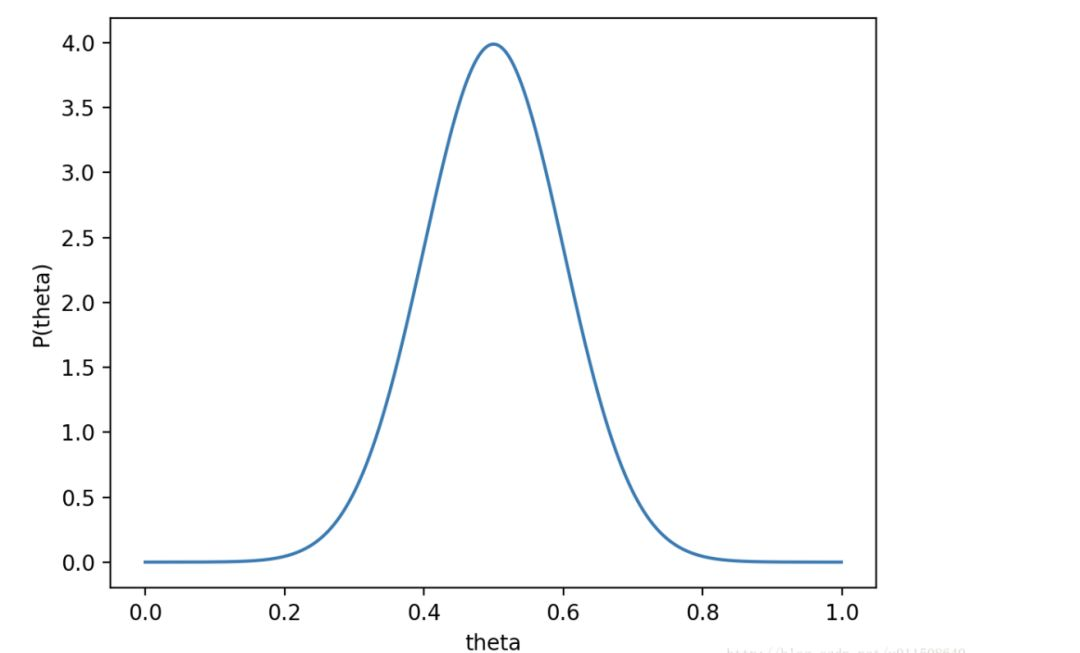则p(x0|theta)p(theta)的函数图像为：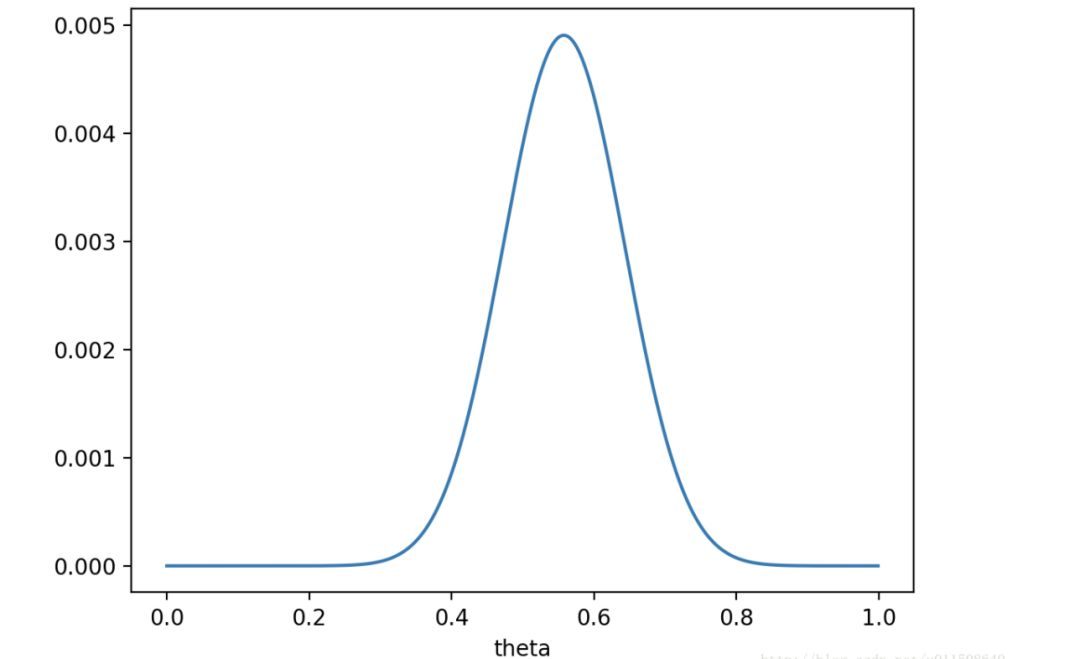注意，此时函数取最大值时，theta取值已向左偏移，不再是0.7。实际上，在theta=0.558时函数取得了最大值。即，用最大后验概率估计，得到theta=0.558。
最后，那要怎样才能说服一个贝叶斯派相信theta=0.7呢？ 你得多做点实验。。
如果做了1000次实验，其中700次都是正面向上，这时似然函数为: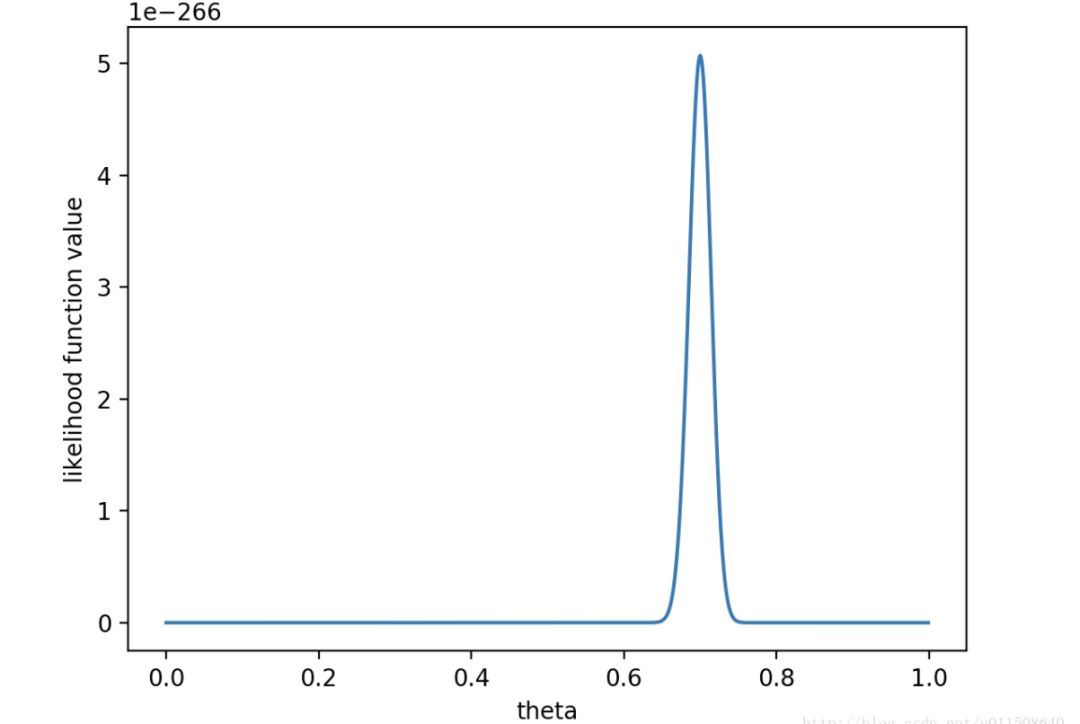如果仍然假设p(theta)为均值0.5，方差0.1的高斯函数，则p(x0|theta)p(theta)的函数图像为：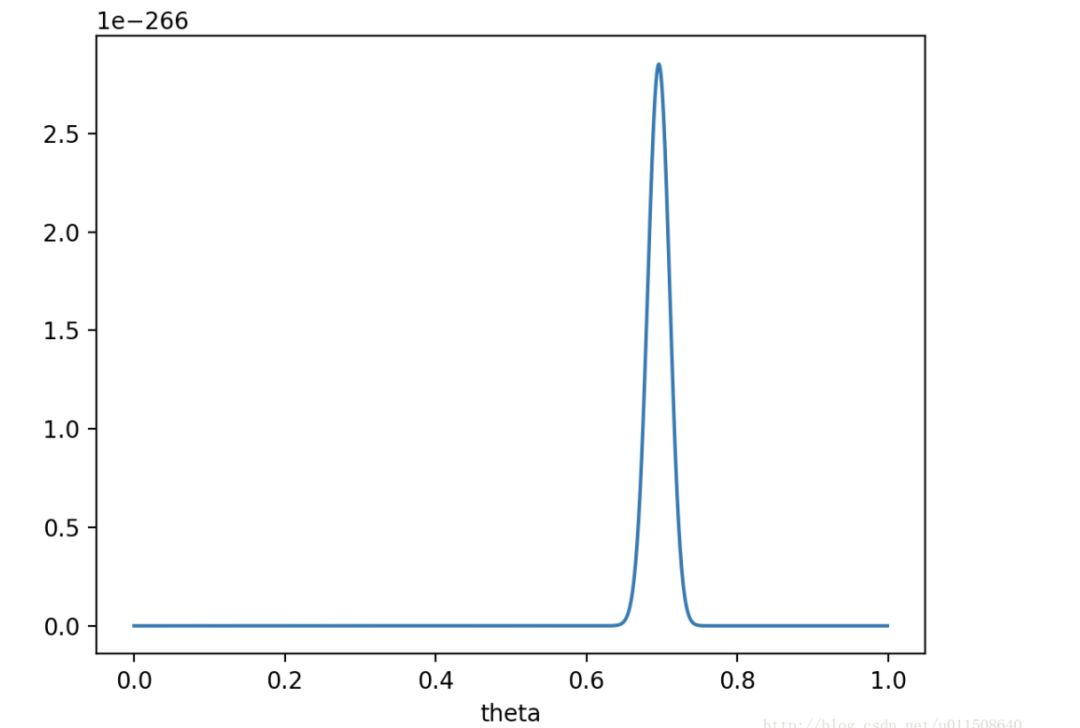在theta=0.696，p(x0|theta)p(theta)取得最大值。
这样，就算一个考虑了先验概率的贝叶斯派，也不得不承认得把theta估计在0.7附近了。
PS. 要是遇上了顽固的贝叶斯派，认为p(theta=0.5)=1，那就没得玩了。。 无论怎么做实验，使用MAP估计出来都是theta=0.5。这也说明，一个合理的先验概率假设是很重要的。（通常，先验概率能从数据中直接分析得到）
2最大似然估计和最大后验概率估计的区别 相信读完上文，MLE和MAP的区别应该是很清楚的了。 MAP就是多个作为因子的先验概率p(theta)。 或者，也可以反过来，认为MLE是把先验概率p(theta)认为等于1，即认为theta为均匀分布，无论theta为何值，p(theta)均为1 文章地址：http://blog.csdn.net/u011508640/article/details/72815981

展开全文MAP
• 本文将入门讲解3个最基本的方法：最大似然估计(Maximum Likelihood Estimation，简称MLE)，最大后验概率估计（Maximum a Posteriori estimation，简称MAP），以及贝叶斯估计。 下面的所有讲解都将用到这样的一组实验...MAP
• 不知看过多少次极大似然估计与最大后验概率估计的区别，但还是傻傻分不清楚。或是当时道行太浅，或是当时积累不够。 这次重游机器学习之路，看到李航老师《统计学习方法》中第一章关于经验风险最小化与结构风险最小...
• 最大似然估计（Maximum likelihood estimation, 简称MLE）和最大后验概率估计（Maximum a posteriori estimation, 简称MAP）是很常用的两种参数估计方法，如果不理解这两种方法的思路，很容易弄混它们。下文将详细...
• Maximum a posteriori estimation（极大后验概率估计）:主题模型
• 在机器学习领域，贝叶斯概率是经常用到的一种模型，而贝叶斯估计中，我们经常会看到两种概率估计模型，一种是最大似然估计，即 Maximum likelihood， 另外一种就是最大后验概率估计，即 Maximum posterior ， 两种...
• 以下所有例子都是抛硬币问题，在两次试验中出现正，反两次结果，求该硬币出现正面的概率p, 最大似然估计： 假设分布为伯努利分布，也就是二项分布，出现正面的概率是p,则下次出现上述...最大后验概率估计： 我们...
• 贝叶斯思想以及与最大似然估计、最大验估计的区别 参数估计（2）：极大似然，最大验，贝叶斯推断以及最大熵 ...机器学习（二十五）— 极大似然估计（MLE）、贝叶斯估计、最大后验概率估计（MAP）区别 ...
• 最大后验概率估计MAP则将θ看作概率分布，欲求使P(X|θ)P(θ)最大的θ。此时，不仅要求P(X|θ)要大，在参数组θ先验分布中θ出现的概率P(θ)也要大，最后求二者共同作用下的最大值。 根据贝叶斯法则+数据集中P(X)...
• 本文在少用数学公式的情况下，尽量仅依靠感性直觉的思考来讲解 极大似然估计 & 极大后验概率估计，并且从名著中找了几个实例给大家看看这两种估计如何应用 & 其非常有趣的特点。
• 从图像降质模型出发，研究运用最大后验概率(MAP)估计法实现图像超分辨率重建。简单介绍了MAP方法的发展现状，并分析了该算法中存在的缺陷，即目标函数的吉布斯(Gibbs)项对于重建图像的噪声抑制力不均衡。针对该缺陷...
• 前言 frequentist statistics：模型参数是未知的定值，观测是随机变量；思想是观测数量趋近于无穷大+真实分布属于模型族中-&...代表是最大后验概率估计MAPE；依赖先验概率 最大似然估计（Ma...机器学习 算法
• 详解最大似然估计（MLE）、最大后验概率估计（MAP），以及贝叶斯公式的理解（1）最大似然估计 这篇文章中提到，关于最大似然估计，使用频率去估计概率，在抛硬币问题中会得到正面向上的概率是0.7的结论，其原因是...
• 与最大似然估计类似，但是最大的不同时，最大后验估计的融入了要估计量的先验分布在其中。故最大后验估计可以看做规则化的最大似然估计。  首先，假设x为独立同分布的采样，θ为模型参数,f为我们所使用的模型。...
• 2 当模型是条件概率分布、损失函数是对数损失函数、模型复杂度由模型的先验概率表示时，结构风险最小化就等价于最大后验概率估计 证明论断1： 极大似然估计：对于观测的随机变量D，其总体分布为 P ( D ; θ...经验风险最小化 结构风险最小化
• 给定观测量x时，关于参数θ的似然函数L(θ)（在数值上）等于给定参数θ变量x的概率： L(θ)=L(θ|x)=pθ=p(x|θ)=p(x;θ)L(\theta) = L(\theta|x)=p_{\theta} = p(x|\theta)=p(x;\theta) 最大似然估计 给定一...MAP 最大似然
• 最大后验概率估计（MAP）1.1 统计1.2 贝叶斯公式(Bayes’ Theorem)1.3 似然函数(likelihood function)和概率函数(probability function)1.5 最大后验概率估计（MAP）2. Wasserstein距离(Wasserstein di
• 李航老师的《统计学习方法》第9页指出“当模型是条件概率分布、损失函数是对数损失函数、模型复杂度由模型的先验概率表示时，结构风险最小化就等价于最大后验概率估计。”下面给出证明，不足不对的地方请指正。 首先...机器学习 结构 正则 优化
• 文章目录似然函数极大似然估计实际应用最大似然估计与极大似然估计(MLE)常见的概率分布模型直观理解 似然函数   在概率论中，设f(x,θ)f(x,\theta)f(x,θ)为总体分布，其中θ\thetaθ为概率分布模型的参数且在...极大似然估计 贝叶斯估计 MAP...